﻿ Introduction to the Bernoulli Function

# An introduction to the Bernoulli function

Peter H. N. Luschny

### Abstract

The Bernoulli function $\operatorname{B}(s, v) = - s\, \zeta(1-s, v)$ interpolates the Bernoulli numbers but can be introduced independently of the zeta function. The point of departure is a modification of the Stieltjes constants based on an integral representation given by J. Jansen. The functional equation of $\operatorname{B}(s, v)$ and its relation to the Riemann $\zeta$ and $\xi$ function is explored.

Classical results of Hadamard, Worpitzky, and Hasse are recast in terms of $\operatorname{B}(s, v).$ The extended Bernoulli function defines the Bernoulli numbers for odd indices harmonizing with rational numbers studied by Euler in 1735 and which are the bridge to the Euler and André numbers. Interpolating functions for both the signed and the unsigned case are given. The Swiss knife polynomials let the integer sequences of the Euler–Bernoulli family calculate easily.

### The question

André Weil recounts the origin of the gamma function in his historical exposition of number theory [66, p. 275]:
Ever since his early days in Petersburg Euler had been interested in the interpolation of functions and formulas given at first only for integral values of the argument; that is how he had created the theory of the gamma function.
The three hundred year success story of Euler's gamma function shows how fruitful this question is. And that the usefulness of such an investigation is not limited by the fact that there are infinitely many ways to interpolate a sequence of numbers.

The question we will explore in this essay is: how can the Bernoulli numbers be interpolated most meaningfully?

### The method

The Bernoulli numbers had been known for some time at the beginning of the 18th century and used in the (now Euler–Maclaurin called) summation formula in analysis, first without realizing that these numbers are the same in each case.

Then, in 1755, Euler baptized these numbers Bernoulli numbers in his Institutiones calculi differentialis (following the lead of de Moivre). After that, things changed, as Edward Sandifer  recounts:

... for once the Bernoulli numbers had a name, their diverse occurrences could be recognized, organized, manipulated and understood. Having a name, they made sense.
The function we are going to talk about is not new. However, it is not treated as a function in its own right and with own name. So we will give the beast a name. We will call the interpolating function the Bernoulli function. If it is true, as Barry Mazur  explains, that the Bernoulli numbers "act as a unifying force, holding together seemingly disparate fields of mathematics," then this should be reflected all the more in this function.

### What to expect

This note is best read as an annotated formula collection, all the proofs are in the references.

The Bernoulli function and the Riemann zeta function are so profoundly interwoven that one's properties can easily be derived from the properties of the other. For instance all the questions Riemann associated with the zeta function can also be discussed with the Bernoulli function.

The introduction of the Bernoulli function leads to greater coherence. It can advise 'generatingfunctionologists' which numbers to hang up on their clothesline (see also this discussion) and in many cases leads to simpler and more natural representations.

Seemingly the first to treat the Bernoulli function very concretely in our sense was J. Jensen , who gave an integral formula for the Bernoulli function of remarkable simplicity. Except for referencing Cauchy's theorem, he did not develop the proof. The proof is worked out in F. Johansson and I. Blagouchine .

### Synopsis

\begin{equation} \operatorname{B}(s,v) \, = \, 2 \pi \int_{- \infty}^\infty \frac{(v-1/2 + i z)^{s}} {({\mathrm{e}}^{- \pi z} + {\mathrm{e}}^{\pi z})^2}\, dz \hspace{67pt} \text{Jensen formula} \nonumber \end{equation} \begin{equation} \operatorname{B}(s) \, = \, \operatorname{B}(s,1) \, = \, -s\, \zeta(1-s) \hspace{83pt} \text{Bernoulli function} \nonumber \end{equation} \begin{equation} \operatorname{B}^c(s) \, = \, \operatorname{B}\left(s,1/2\right) \hspace{95pt} \text{Central Bernoulli function} \nonumber \end{equation} \begin{equation} \operatorname{B}^{*}(s) \, = \, \operatorname{B}(s)(1-2^s) \hspace{61pt} \text{Alternating Bernoulli function} \nonumber \end{equation} \begin{equation} \operatorname{B}_n \, = \, \operatorname{B}(n) \hspace{168pt} \text{Bernoulli numbers} \nonumber \end{equation} \begin{equation} \operatorname{G}(s,v) \, = \, 2^s \left( \operatorname{B}\left(s,\frac{v}{2}\right) - \operatorname{B}\left(s,\frac{v+1}{2}\right) \right) \hspace{34pt} \text{Genocchi function} \nonumber \end{equation} \begin{equation} \operatorname{G}(s) \, = \, \operatorname{G}(s,1)\,=\, 2^s \left( \operatorname{B}^{c}(s) - \operatorname{B}(s) \right) \hspace{58pt} \text{Genocchi function} \nonumber \end{equation} \begin{equation} \operatorname{E}(s,v) \, = \, - \frac{\operatorname{G}(s+1,v)}{s+1} \hspace{72pt} \text{Generalized Euler function} \nonumber \end{equation} \begin{equation} \operatorname{E}(s) \, = \, \operatorname{E}(s,1) \hspace{174pt} \text{Euler function} \nonumber \end{equation} \begin{equation} \operatorname{E}^{c}(s) \, = \, 2^{s} \operatorname{E}(s,\tfrac{1}{2}) \hspace{117pt} \text{Central Euler function} \nonumber \end{equation} \begin{equation} \operatorname{E}_n \, = \, \operatorname{E}^{c}(n) \hspace{185.5pt} \text{Euler numbers} \nonumber \end{equation} \begin{equation} \widetilde{\zeta}(s) \, = \, \zeta \left({s} \right) + \frac{ \zeta \left({s},{\frac{1}{4}} \right) - \zeta \left({s},{\frac{3}{4}} \right) } {2 ^{s} - 2} \hspace{44pt} \text{Extended zeta function} \nonumber \end{equation} \begin{equation} \mathcal{B}(s) \, = \, -s\, \widetilde{\zeta}(1-s) \hspace{87pt} \text{Extended Bernoulli function} \nonumber \end{equation} \begin{equation} \mathcal{E}(s) \, = \, \frac{4^{s+1} - 2^{s+1}}{ (s+1)!} \mathcal{B}(s+1) \hspace{66pt} \text{Extended Euler function} \nonumber \end{equation} \begin{equation} \mathcal{A}(s) \, = \, s! \, \mathcal{E}(s) = \, (2^{s+1} - 4^{s+1})\, \widetilde{\zeta}(-s) \hspace{70pt} \text{André function} \nonumber \end{equation} \begin{equation} \mathcal{A}^{\star} (s) \, = \, i (i^s \operatorname{Li}_{-s}(-i) - (-i)^s \operatorname{Li}_{-s}(i)) \hspace{17pt} \text{Unsigned André function} \nonumber \end{equation} \begin{equation} \mathcal{B}^{\star} (s) \, = \frac{s\, \mathcal{A}^{\star} (s-1)}{4^s - 2^s} \hspace{33pt} \text{Unsigned extended Bernoulli function} \nonumber \end{equation} \begin{equation} \operatorname{\xi}(s) \, = \, \operatorname{B}(s)\, \frac{\sigma !}{\pi^{\sigma}} \ \ \text{with } \sigma = (1-s)/2 \hspace{49pt} \text{Riemann }\xi\text{ function} \nonumber \end{equation} \begin{equation} \gamma \, = \, - \operatorname{B}^{'}(0) \ \text{(Euler),} \quad \gamma_{n-1} \, = \, - \frac{1}{n}\operatorname{B}^{(n)}(0) \quad \hspace{15pt} \text{Stieltjes constants} \nonumber \end{equation}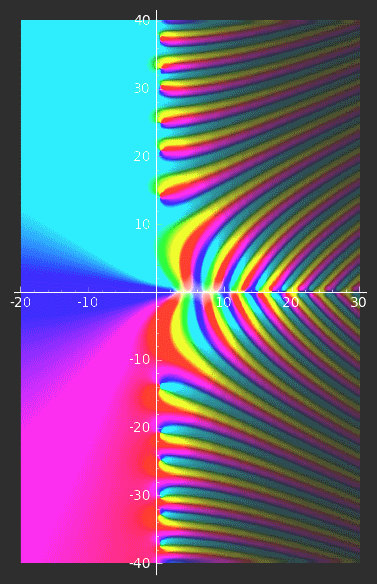### The Stieltjes constants

The generalized Euler constants, also called Stieltjes constants, are the real numbers $\gamma_n$ defined by the Laurent series in a neighborhood of $s=1$ of the Riemann zeta function ([Riemann], [DLMF]). \begin{equation} \zeta(s)= \frac{1}{s-1}+\sum_{n=0}^\infty \frac{(-1)^n}{n!} \gamma_n (s-1)^n, \qquad (s \ne 1) . \label{q1} \end{equation} As a special case they include the Euler constant $\gamma_0 = \gamma \ \, ( \approx \frac{228}{395})$. An extensive discussion with many historical notes can be found in . For the numerical values consult . I. V. Blagouchine  following J. Franel  shows that \begin{equation} \gamma_n \,=\, - \frac{2\, \pi}{n+1} \int\limits_{- \infty}^{+\infty}\, \frac{ \log\!\left(\frac{1}{2} + iz \right)^{n+1}} {\left( {{\mathrm{e}}^{- \pi \,z}}+{{\mathrm{e}}^{\pi \,z }} \right) ^{2}}\,{dz} . \label{q2} \end{equation} Recently it has been observed that the integral representation (\ref{q2}) can be employed in a particularly efficient way to numerically approximate the Stieltjes constants with prescribed precision .

### The Bernoulli constants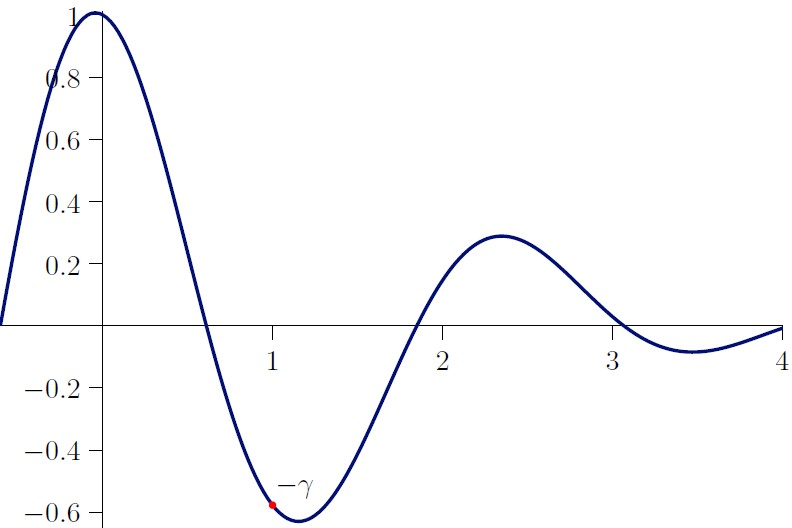The Bernoulli constants seen as values of a real function. The Bernoulli constants $\beta_n$ are defined for $n \ge 0$ as

\begin{equation} \beta_n \, = \, 2\pi \! \int\limits_{- \infty }^{+\infty }{\frac { \log\! \left( \frac{1}{2} + iz \right) ^n } {\left( {{\mathrm{e}}^{- \pi \,z}}+{{\mathrm{e}}^{\pi \,z }} \right) ^{2}}}\,{dz} . \label{q3} \end{equation}

We write $f(x)^n$ for $(f(x))^n$ and take the principal value for the logarithm implicit in the exponential, here and in all later similar formulas.

The index $n$ in (\ref{q3}) is not restricted to integer values. For illustration the function $\beta_r$ is plotted in the figure above, where the index $r$ of $\beta$ is understood to be a real number. The table below displays some numerical values of Bernoulli constants.

 $r$ $\beta(r)$ $- 1$ -1.0967919991262275651322398023421657187190 $\dots$ $- 1/2$ 0.3000952439768656513643742483305378454480 $\dots$ $0$ 1 $1/2$ 0.2364079388130323148951169845913737350793 $\dots$ $1$ -0.5772156649015328606065120900824024310421 $\dots$ $3/2$ -0.4131520868458801199329318166967102536980 $\dots$ $2$ 0.1456316909673534497211727517498026382754 $\dots$ $5/2$ 0.2654200629584708272795102903586382709016 $\dots$ $3$ 0.0290710895786169554535911581056375880771 $\dots$ $7/2$ -0.0845272473711484887663180676975841853310 $\dots$ $4$ -0.0082153376812133834646401861710135371428 $\dots$

### The Bernoulli function

The Bernoulli function is defined as \begin{equation} \operatorname{B}(s) \, = \, \sum _{n=0}^{\infty }\beta_{n} \frac {s^n}{n!}\,. \label{Bdef} \end{equation} Note that $\beta_0 = 1$, thus in particular $\operatorname{B}(0) = 1$. From (\ref{q2}) and (\ref{q3}) we see that $\gamma_n = - \beta_{n+1}/(n+1)$. Thus we get: \begin{align} \operatorname{B}(s) & = \sum _{n=0}^{\infty }\beta_{n} \frac {s^n}{n!} \nonumber \\ &= 1+ \sum _{n=1}^{\infty } \beta_{n} \frac{s^n}{n!} \nonumber \\ &= 1+ \sum _{n=0}^{\infty } \beta_{n+1} \frac{s^{n+1}}{(n+1)!} \nonumber \\ &= 1 - \sum_{n=0}^\infty \gamma_n \frac{s^{n+1}}{n!} \nonumber \\ &= 1 - s \sum_{n=0}^\infty \gamma_n \frac{s^n}{n!} \nonumber \\ &=-s\zeta(1-s) . \label{Bzeta} \end{align} Here the singularity of $-s\zeta(1-s)$ at $s=0$ is removed by $\operatorname{B}(0) = 1$. So $\operatorname{B}(s)$ is an entire function with its defining series converging everywhere in $\mathbb{C}.$

### The expansion of the Bernoulli function

The Bernoulli function $\operatorname{B}(s) = -s\zeta(1-s)$ can be expanded by using the generalized Euler–Stieltjes constants \begin{equation} \operatorname{B}(s)\, =\, 1 - \gamma s - \gamma_1 s^2 - \frac{\gamma_2}{2} s^3 - \frac{\gamma_3}{6} s^4 \,\ldots \ , \label{q7} \end{equation} or in its more natural form using the Bernoulli constants

\begin{equation} \operatorname{B}(s) \, = \, 1 + \beta_1 s + \frac{\beta_2}{2} s^2 + \frac{\beta_3}{6} s^3 + \frac{\beta_4}{24} s^4 \,\ldots \ . \label{q8} \end{equation}

Although we will always refer to the well-known properties of the zeta function when using (\ref{Bzeta}), our definition of the Bernoulli function and the Bernoulli numbers only depends on (\ref{q3}) and (\ref{Bdef}).

### The Bernoulli numbers

We define the Bernoulli numbers as the values of the Bernoulli function at the nonnegative integers. According to (\ref{Bdef}) this means \begin{equation} \operatorname{B}_n \, = \, \operatorname{B}(n) \, = \, \sum _{j=0}^{\infty }\beta_{j} \frac {n^j}{j!}. \label{bernum} \end{equation} Since for $n > 1$ an odd integer $-n\zeta(1-n) = 0$, the Bernoulli numbers vanish at these integers and $\operatorname{B}_{n} = 1 - n \sum_{j=0}^\infty \gamma_j \frac{n^j}{j!}$ implies \begin{equation} \sum_{j=0}^\infty \gamma_j \frac{n^j}{j!} = \frac{1}{n} \text{ and } \sum_{j=0}^\infty \beta_j \frac{n^j}{j!} = 0 \qquad (n > 1 \text{ odd}). \label{a1} \end{equation}

### Integral formulas for the Bernoulli constants

Let us come back to the definition of $\beta_n$ as given in (\ref{q3}). The appearance of the imaginary unit forces complex integration; on the other hand, only the real part of the result is used. Fortunately, the definition can be simplified such that the computation stays in the realm of reals provided $n$ is a nonnegative integer.

Using the symmetry of the integrand with respect to the $y$-axis and $\left( {{\mathrm{e}}^{- \pi \,z}} + {{\mathrm{e}}^{\pi \,z }} \right) ^{2} = 4 \cosh(\pi z)^2$ we get from the definition (\ref{q3})

\begin{equation} \beta_n \ = \ \pi \! \int_{0}^{\infty}\, {\frac{\Re\! \left(\log(\frac12 + iz)^n \right) }{ \cosh(\pi z) ^2 }} dz \, . \label{q9} \end{equation} For the numerator of the integrand we set for $n \ge 0$ \begin{equation} \sigma_n(z) \, = \, \Re (\log ( \tfrac{1}{2} + iz )^n ). \label{q10} \end{equation} By induction we see that \begin{equation} \sigma_n(z) = \sum_{ k = 0 }^{ \lfloor n/2 \rfloor } (-1)^{k} \binom{n}{2k} a(z)^{n - 2k}\, b(z)^{2 k}, \label{q11} \end{equation} where $a(z) = \log(z^2+ \frac{1}{4})/2$ and $b(z) = \arctan(2z)$.

Therefore the constants can be computed by real integrals,

\begin{equation} \beta_n \,=\, \pi \! \int_{0}^{\infty} \frac{\sigma_n(z)}{\cosh(\pi z)^2}\, dz \, . \label{q12} \end{equation} Similarly for the Stieltjes constants, \begin{equation} \gamma_n \,=\, - \frac{\pi}{n+1} \int_{0}^{\infty} \frac{\sigma_{n+1}(z)}{\cosh(\pi z)^2}\, dz \,. \label{q13} \end{equation}

### Some special integral formulas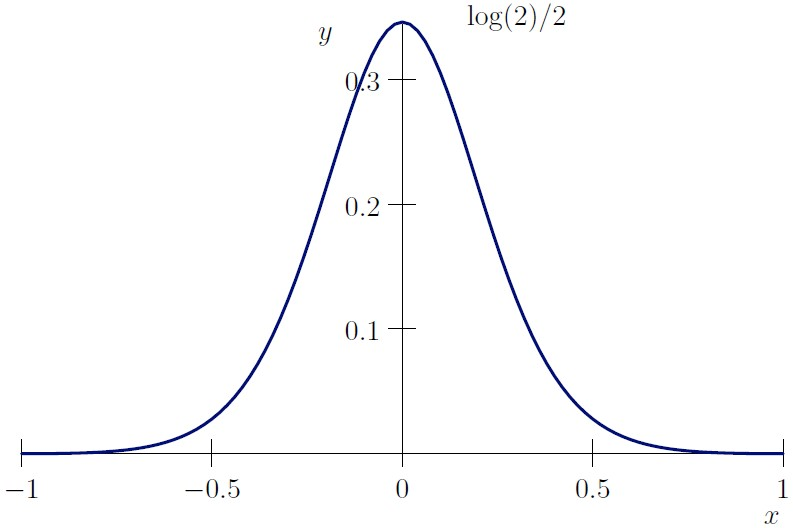Integral representation of $\gamma/\pi$. Formula (\ref{q13}) reads for $n=0$ \begin{equation} \gamma \, =\, - \pi \int_{0}^{\infty} \frac{\log(z^2+ \tfrac{1}{4} ) }{2 \cosh(\pi z)^2} \, dz . \label{q14} \end{equation} This follows since for real $z$ \begin{equation} \sigma_1(z) = \Re \log\left(\frac12+i z\right) = \log\left(\frac12\sqrt{4 z^2 + 1}\right) = \frac12\log\left(z^2+\frac14\right). \nonumber \end{equation} Using the symmetry of the integrand with respect to the $y$-axis this can be rephrased as: Euler's gamma is $\pi$ times the integral of $- \log((z^2+ \tfrac{1}{4} )^{\tfrac{1}{4}})\mathrm{sech}(\pi z)^2$ over the real line (see the figure above). The constant ${\gamma} / { \pi}$ is A301813 in the OEIS.

Using the abbreviations $a = \log(z^2+ \frac{1}{4})/2, \, b = \arctan(2z)$ and $c = \cosh(\pi z)$ the first few Bernoulli constants are by (\ref{q11}):

\begin{align} \beta_1 \, &=\, \pi \int_{0}^{\infty} \frac{a}{c^2} \, dz \,, \label{q15} \\ \beta_2\, &=\, \pi \int_{0}^{\infty} \frac{a^2 - b^2}{ c^2} \,dz \,, \label{q16} \\ \beta_3\, &=\, \pi \int_{0}^{\infty} \frac{a^3 - 3 a b^2 }{ c^2} \,dz \,, \label{q17} \\ \beta_4\, &=\, \pi \int_{0}^{\infty} \frac{a^4 - 6 a^2b^2 + b^4}{c^2} \,dz \,, \label{q18} \\ \beta_5\, &=\, \pi \int_{0}^{\infty} \frac{a^5 - 10 a^3b^2 + 5ab^4}{c^2} \,dz \,. \label{q19} \end{align}

### Generalized Stieltjes and Bernoulli constants

We recall that $\gamma_n/n!$ is the coefficient of $(1-s)^n$ in the Laurent expansion of $\zeta(s)$ about $s = 1$ and $\gamma_n(v)/n!$ is the coefficient of $(1-s)^n$ in the Laurent expansion of $\zeta(s,v)$ about $s = 1$. In other words, with the generalized Stieltjes constants $\gamma_n(v)$ we have the Hurwitz zeta function $\zeta(s,v)$ in the form \begin{equation} \zeta(s,v)=\frac{1}{s-1}+\sum_{n=0}^\infty \frac{(-1)^n}{n!} \gamma_n(v) (s-1)^n, \quad(s \neq 1). \label{q35} \end{equation} The generalized Stieltjes constants may be computed for $n \ge 0$ and $\Re(v) > \frac12$ by an extension of the integral representation (\ref{q2}), see [34, formula 2]. \begin{equation} \gamma_n(v) \, = \, - \frac{\pi}{2(n+1)} \int_{- \infty}^\infty\frac{\log\!\left(v- \tfrac{1}{2} + i z\right) ^{n+1}} {\cosh(\pi x)^2}\, dz. \label{int333} \end{equation} The generalized Bernoulli constants are defined as

\begin{equation} \beta_s(v) = 2 \pi \!\int_{- \infty}^{+\infty} \!\frac{\, \log\!\big(v - \frac12 + ix\big) ^s \,}{ \left( {\mathrm{e}}^{- \pi x} + {\mathrm{e}}^{ \pi x} \right)^2 }\,dx. \label{q37} \end{equation}

Note that $\beta_{0}(v) = 1$ for all $v$ and $\beta_{n}(v) = - n \gamma_{n-1}(v)$ for $n \ge 1$.

### The generalized Bernoulli function

Next we introduce the generalized Bernoulli function $\operatorname{B}(s, v),$ which is the analog of the Hurwitz zeta function. The new parameter $v$ can be any complex number which is not a non-positive integer. The generalized Bernoulli function is defined as

\begin{equation} \operatorname{B}(s,v) \, = \, \sum _{n=0}^{\infty }\beta_{n}(v) \frac {s^n}{n!}\, . \label{Generalizedgamma} \end{equation}

For $v=1$ this is the ordinary Bernoulli function (\ref{Bdef}). Using the identities from the last section we get \begin{equation} \operatorname{B}(s, v) = 1 - s \sum_{n=0}^{\infty} \gamma_n(v) \frac{s^n}{n!} . \label{q36a} \end{equation} Thus the generalized Bernoulli function can be represented by \begin{equation} \operatorname{B}(s, v) = - s \, \zeta(1-s, v), \qquad (s \ne 1). \label{q36} \end{equation} This also embeds the Bernoulli polynomials as \begin{equation} \operatorname{B}_{n}(x) \,=\, \operatorname{B}(n, x) \quad (n \ge 0, n \text{ integer}) . \label{q22} \end{equation} This follows from (\ref{q36}) (see for instance [4, Th. 12.13]) and the fact that $\operatorname{B}(0, x) \, = \, 1.$

### Integral formulas for the Bernoulli function

The integral formulas for the Bernoulli constants can be transferred to the Bernoulli function itself. In the first step we reproduce a formula by J. L. Jensen, which he gave in a reply to E. Cesàro in the L'Intermédiaire des mathématiciens.

\begin{equation} (s-1)\zeta(s) \, = \, 2 \pi \int_{- \infty}^\infty\frac{( \tfrac{1}{2} +iy)^{1-s}}{({\mathrm{e}}^{\pi y} + {\mathrm{e}}^ {- \pi y})^2}\, dy. \label{int1} \end{equation}

"... [this formula] is remarkable because of its simplicity and can easily be demonstrated with the help of Cauchy's theorem."
How Jensen actually computed $(s-1)\zeta(s)$ is unclear, since the formula for the coefficients $\operatorname{c}_v$, which he states, rapidly diverges. This was observed by V. Kotěšovec (personal communication).

In a numerical example Jensen uses the Bernoulli constants in the form $\operatorname{c}_v \, = \, (-1)^v \beta_v/ {v!}.$ Applied to the Bernoulli function, Jensen's formula is written as

\begin{equation} \operatorname{B}(s) \, = \, 2 \pi \int_{- \infty}^\infty\frac{( \tfrac{1}{2} +iz)^{s}} {({\mathrm{e}}^{\pi z} + {\mathrm{e}}^ {- \pi z})^2}\, dz. \label{int4} \end{equation}

This formula can be seen as a special case of the first formula in theorem 1 in Johansson and Blagouchine , [61, p. 92], .

P. Hadjicostas remarks that from this theorem also the corresponding representation for the generalized Bernoulli function can be derived:

For all $s \in \mathbb{C}$ and $v \in \mathbb{C}$ with $\Re(v) \ge 1/2$

\begin{equation} \operatorname{B}(s, v) \, = \, 2 \pi \int_{- \infty}^\infty\frac{(v- \tfrac{1}{2} + i z)^{s}} {({\mathrm{e}}^{- \pi z} + {\mathrm{e}}^ {\pi z})^2}\, dz. \label{int5} \end{equation}

### The Hurwitz–Bernoulli function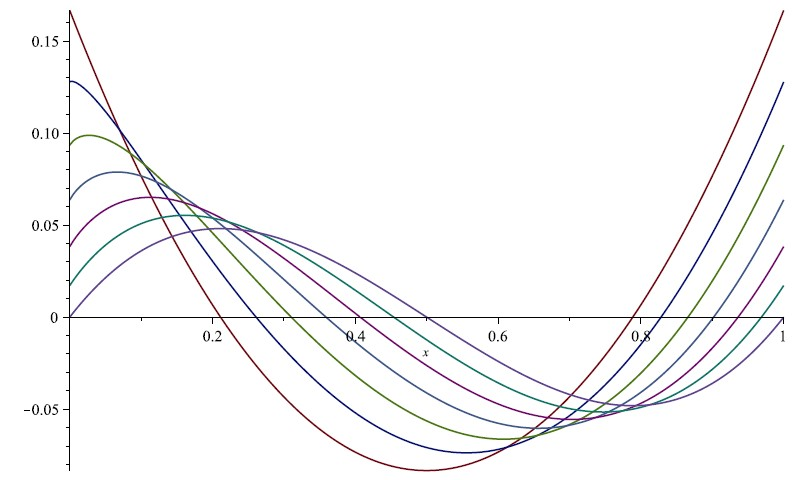The Hurwitz–Bernoulli functions with $s = 2 + k/6$ for $0 \le k \le 6$ deform $\operatorname{B}_{2}(x)$ into $\operatorname{B}_{3}(x).$ The Hurwitz–Bernoulli function is defined as

\begin{align} \operatorname{H}(s, v) &= {\mathrm{e}}^{-i \pi s/2}\, \operatorname{L}(s, v) + {\mathrm{e}}^{i \pi s/2} \, \operatorname{L}(s, 1-v), \label{q23} \\ \operatorname{L}(s, v) &= - \frac{s\, !}{(2 \pi)^s} \operatorname{Li}_s({\mathrm{e}}^{2 \pi i v}). \label{bLi} \end{align}

Here $\operatorname{Li}_s(v)$ denotes the polylogarithm. The proposition that \begin{equation} \operatorname{B}_{s}(v) =\operatorname{B}(s, v) = \operatorname{H}(s, v), \ \ \text{for } 0 \le v \le 1 \text{ and } s > 1, \label{HurwitzFormula} \end{equation} goes back to Adolf Hurwitz. In the corresponding case for the zeta function (\ref{HurwitzFormula}) is known as the Hurwitz formula [4, p. 71].

With the Hurwitz–Bernoulli function the Bernoulli polynomials can be continuously deformed into each other (see the figure above).

### The central Bernoulli function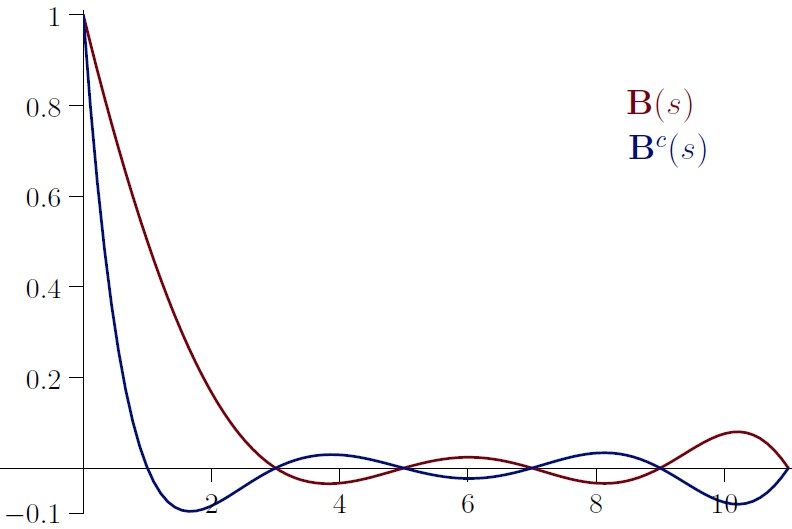The Bernoulli function and the central Bernoulli function. Setting $v=1$ in (\ref{HurwitzFormula}) the Hurwitz–Bernoulli function simplifies to

\begin{equation} \operatorname{B}_{s}(1) \, =\, -2\, s! \operatorname{Li}_s(1) \cos(s \pi /2)/ (2 \pi)^{s}, \quad (s>1). \label{bs1} \end{equation}

For $s>1$ one can replace the polylogarithm by the zeta function and then apply the functional equation of the zeta function to get \begin{equation} \operatorname{B}_{s}(1) \, =\, -s \zeta(1-s), \qquad (s>1). \nonumber \end{equation} Thus the Bernoulli function is a vertical section of the Hurwitz–Bernoulli function, $\operatorname{B}(s) = \operatorname{B}_{s}(1),$ similarly as the Bernoulli numbers are special cases of the Bernoulli polynomials, $\operatorname{B}_n = \operatorname{B}_{n}(1)$.

Setting $v=1/2$ in the Hurwitz–Bernoulli function leads to a second noteworthy case. Then (\ref{HurwitzFormula}) reduces to

\begin{equation} \operatorname{B}_{s}(1/2) \, =\, -2\, s! \operatorname{Li}_s(-1) \cos(s \pi /2)/ (2 \pi)^{s}, \quad (s>1). \label{bs12} \end{equation}

For $s>1$ we can replace the polylogarithm by the negated alternating zeta function. We call $\operatorname{B}^{c}(s) = \operatorname{B}_{s}(1/2)$ the central Bernoulli function. $\operatorname{B}^{c}(s)$ has the same trivial zeros as the Bernoulli function, plus a zero at the point $s=1$. (See the plot above.)

An integral representation for the central Bernoulli function follows from (\ref{int5}). For all $s \in \mathbb{C}$

\begin{equation} \operatorname{B}^{c}(s) \, = \, 2 \pi \int_{- \infty}^\infty\frac{(i z)^{s}} {({\mathrm{e}}^{- \pi z} + {\mathrm{e}}^ {\pi z})^2}\, dz. \label{intcenter5} \end{equation}

### The central Bernoulli polynomials

The central Bernoulli numbers are defined as \begin{equation} \operatorname{B}^{c}_n = 2^n \operatorname{B}_{n}(1/2). \label{CBN} \end{equation} The first few are, for $n \ge 0$: \begin{equation} 1,\, 0,\, - \frac{1}{3},\, 0,\, \frac{7}{15},\, 0,\, - \frac{31}{21},\, 0,\, \frac{127}{15}, \, 0,\, - \frac{2555}{33}, \, 0, \frac{1414477}{1365}, \ldots . \nonumber \end{equation} Unsurprisingly Leonhard Euler in 1755 in his Institutiones also calculated some central Bernoulli numbers B(3,1) and B(5,1) (Opera Omnia, Ser. 1, Vol. 10, p. 351).

The central Bernoulli polynomials are defined, like the Bernoulli polynomials, as an Appell sequence.

\begin{equation} \operatorname{B}_n^{c}(x) \, = \, \sum_{k=0}^n \binom{n}{k} \operatorname{B}^{c}_k x^{n-k}. \end{equation} The parity of $n$ equals the parity of $\operatorname{B}_n^{c}(x)$ (the Bernoulli polynomials do not possess this property).
 $\phantom{-}1$ $\phantom{-} x$ $-{\frac{1}{3}}\,+\,{x}^{2}$ $-{x}\,+\,{x}^{3}$ $\phantom{-}{\frac{7}{15}}\,- \,{2\, {x}^{2}}\,+\,{x}^{4}$ $\phantom{-}{\frac {7}{3}\,x}\,- \,{\frac {10}{3}\,{x}^{3}}\,+\,{x}^{5}$ $-{\frac{31}{21}}\,+\,{7\,{x}^{2}}\,- \,5\,{x}^{4}\,+\,{x}^{6}$ $-{\frac{31}{3}\,x}\,+\,{\frac {49}{3}\,{x}^{3}}\,- \,7\,{x}^{5}\,+\,{x}^{7}$ ${\frac{127}{15}}-{\frac {124}{3}\,{x}^{2}}+{\frac {98}{3}\,{x}^{4}}-{\frac {28}{3}\,{x}^{6}}+{x}^{8}$ ${\frac{381}{5}\,x}-124\,{x}^{3}+{\frac {294}{5}\,{x}^{5}}-12\,{x}^{7}+{x}^{9}$
Despite their systematic significance the central Bernoulli polynomials were not in the OEIS database at the time of writing these lines (now they are filed in A335953).

In the context of 'going halves' also the following identity is worth noting (we will come back to it later):

\begin{equation} 2^n \operatorname{B}_n(1) \, = \, \sum_{k=0}^n \binom{n}{k} 2^{k} \operatorname{B}_k\left( \tfrac{1}{2} \right). \label{bernoulligoeshalf} \end{equation}

### The Genocchi function

How much does the central Bernoulli function deviate from the Bernoulli function? The Genocchi function gives an answer to this (up to a scaling factor). \begin{equation} \operatorname{G}(s) \, = \, 2^s \left( \operatorname{B}_{s}( \tfrac{1}{2} ) - \operatorname{B}_{s}(1) \right) . \label{GenocciFun} \end{equation} From the identities (\ref{bs1}) and (\ref{bs12}), it follows that the Genocchi function can be represented, first for $s>1$ and then by analytical continuation in general, as \begin{equation} \operatorname{G}(s) \, = \, 2 (1 - 2^s) \operatorname{B}(s). \label{GenocciFun2} \end{equation} A useful property of the Genocchi function is that it takes integer values for non-negative integer arguments. The $\operatorname{G}_n = \operatorname{G}(n)$ are known as Genocchi numbers (A226158).

The Genocchi polynomials are defined as

\begin{equation} \operatorname{G}_{n}(x) \, = \, 2^n \left( \operatorname{B}_n\left(\frac{x}{2}\right) - \operatorname{B}_n\left( \frac{x+1}{2}\right) \right). \label{q123} \end{equation}

They describe the difference between the Bernoulli polynomials and the central Bernoulli polynomials, up to a scaling factor. The integer coefficients of these polynomials are recorded as A333303 in the OEIS. The Genocchi function is closely related to the alternating Bernoulli function as we will see later.

### Derivatives of the Bernoulli function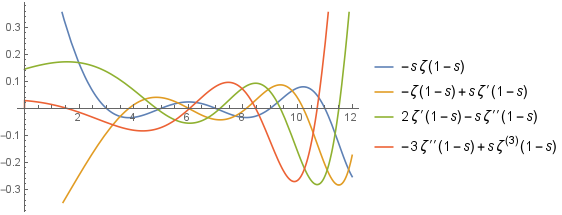The derivatives of the Bernoulli function $\operatorname{B}^{(n)}(s),\, 0 \le n \le 3.$

The Bernoulli constants are related to the derivatives of the Bernoulli function. With the Riemann zeta function we have

\begin{equation} \operatorname{B}^{(n)}(s) = (-1)^n \left( n \zeta^{(n-1)}(1-s) - s \zeta^{(n)}(1-s) \right). \label{q25} \end{equation}

Here $\operatorname{B}^{(n)}(s)$ denotes the $n$-th derivative of the Bernoulli function. Taking $\lim_{s \rightarrow 0}$ on the right hand side of (\ref{q25}) we get \begin{equation} \operatorname{B}^{(n)}(0) = \beta_n = - n \, \gamma_{n-1} \quad ( n \ge 1) \, . \label{q26} \end{equation} The $n$-th derivative of the Hurwitz $\zeta$-function with respect to $s$ is \begin{equation} \zeta^{(n)}(s, v) = \frac{\partial^n}{\partial s^n}\, \zeta(s, v) \label{q27} \end{equation} and the $n$-th derivative of the generalized Bernoulli function is \begin{equation} \operatorname{B}^{(n)}(s, v) = (-1)^n \, (n \, \zeta^{(n-1)}(1-s, v) - s\, \zeta^{(n)}(1-s, v)) , \label{q28} \end{equation} using the limiting value if $s=0$. Rewriting (\ref{q28}) we have for $n \ge 1$

\begin{equation} \frac{\gamma_{n}(v)}{\Gamma(v)} = (-1)^n \lim_{s \rightarrow 0} \left( n\, \zeta^{(n-1)}(1-s,v) - s\, \zeta^{(n)}(1-s,v) \right) . \label{q32} \end{equation}

Comparing this with the representation of the Stieltjes constants we see that $\gamma_n(1) = \gamma_{n}$ and that relation (\ref{q26}) generalizes to \begin{equation} \operatorname{B}^{(n)}(0, n ) = - n \, \gamma_{n-1}(n) \qquad ( n \ge 1) \, . \label{q33} \end{equation} The plot below summarizes much of what has been said: it shows the Bernoulli function together with the Bernoulli numbers and the additive inverse of the derivative of the Bernoulli function which hits Euler's $\gamma$ at the origin.

Entire books  have been written about the emergence of Euler's gamma in number theory. The identity ${- \operatorname{B}'(0) = \gamma }\label{q39}$ is one of the beautiful places where this manifests.$- \operatorname{B}'(s)$ hits Euler's $\gamma$ at $s=0,$ red points are Bernoulli numbers

### The logarithmic derivative

The logarithmic derivative of a function $\operatorname{F}$ will be denoted by \begin{equation} \mathcal{L}\!\operatorname{F}(s) = \frac{ \operatorname{F}'(s)}{ \operatorname{F}(s)}. \nonumber \end{equation} In particular we will write $\mathcal{L}\!\operatorname{B}(s),\, \mathcal{L}\zeta(s)$ and $\mathcal{L}\Gamma(s)$ for the logarithmic derivative of the Bernoulli function, the $\zeta$ function and the $\Gamma$ function (also known as digamma function $\psi$).

We will also use the notation $\rho(s)$ for the function

\begin{equation} \rho(s) \, =\, \frac{1}{s} + \frac{\pi}{2} \cot(s) - \log(2\pi). \nonumber \end{equation} In terms of the zeta function $\mathcal{L}\!\operatorname{B}(s)$ can also be written \begin{equation} \mathcal{L}\!\operatorname{B}(s) \,=\, \frac{1}{s} - \mathcal{L}\zeta(1-s). \label{ws2} \end{equation} The case $s = \frac12$ is particularly interesting. It is known that the truth of the proposition \begin{equation} \mathcal{L}\!\operatorname{B}\left(\frac12\right) + 2 \, = \, - \frac{2}{\pi}\int_0^{\infty} \log\left|\frac{\zeta(\frac{1}{2}+it)} {\zeta(\frac{1}{2})}\right|\ \frac{dt}{t^2} \end{equation} is equivalent to the Riemann hypothesis (see [30, Th. 7.26]). Note that $\mathcal{L}\!\operatorname{B}(\frac12) \, = 2 - \pi/4 - \gamma/2 - \log(8 \pi)/2,$ (cf. also A335263).

From (\ref{ws2}) we can infer, by well known relations (see ),

\begin{equation} \mathcal{L}\!\operatorname{B}(s) \,=\, \mathcal{L}\Gamma(s) + \mathcal{L}\zeta(s) + \rho(s) . \label{BZ} \end{equation}

Expressing the $\zeta$ function by the Euler product, we can derive from (\ref{BZ}) and the von Mangoldt's function $\Lambda$ for $\Re(s) > 1$, \begin{equation} \sum_{n=1}^{\infty} \frac{\Lambda(n)}{n^{s}} \, = \, \mathcal{L}\Gamma(s) - \mathcal{L}\!\operatorname{B}(s) + \rho(s). \end{equation}

### Bernoulli cumulants

The series expansion of $\mathcal{L}\!\operatorname{B}(s) = \sum_{n = 1}^{\infty} b_n s^{n-1}$ at $s = 0$ starts \begin{align*} &\mathcal{L}\!\operatorname{B}(s) = \beta_{{1}} +( \beta_{{2}} - {\beta_{{1}}}^{2} ) \,s +( \beta_{{3}} - 3\,\beta_{{1}}\beta_{{2}} + 2\,{\beta_{{1}}}^{3} ) \,s^2/2 \\ &+ ( \beta_{{4}} -3\, {\beta_{{2}}}^{2} -4\,\beta_{{1}}\beta_{{3}} +12\,{\beta_{{1}}}^{2}\beta_{{2}} -6\,{\beta_{{1}}}^{4} ) \,s^3/6 + O(s^4). \label{q41} \end{align*} The coefficients $b_n$ are given by \begin{equation} b_n = [ s^n ] \log \bigg( \sum_{n = 0}^{\infty} \beta_n \frac{s^n}{n!} \bigg). \label{q42} \end{equation} In other words, the coefficients are the logarithmic polynomials generated by the Bernoulli constants (Comtet [9, p.140]). These polynomials may be called Bernoulli cumulants, following a similar naming by A. Voros [65, 3.16].

The numerical values appearing in this expansion, listed as an irregular triangle, are A263634, row-reversed:

 $1$ $1$ $-1$ $1$ $-3$ $2$ $1$ $[- 3,\, -4]$ $12$ $-6$ $1$ $[-10,\, -5]$ $[30,\, 20]$ $-60$ $24$ $1$ $[-10,\, -15,\, -6]$ $[30,\, 120,\, 30]$ $[-270,\, -120]$ $360$ $-120$

### Worpitzky numbers

The numbers in the above triangle are refinements (indicated by the square brackets) of the signed Worpitzky numbers $\operatorname{W}(n,k)$ (, , A163626, A028246). \begin{equation} \operatorname{W}(n,k) \,= \, (-1)^k k! \genfrac\{\}{0pt}{}{n+1}{k+1}. \label{q43} \end{equation} Here $\genfrac\{\}{0pt}{}{n}{k}$ denotes the Stirling set numbers. Generalizations based on Joffe's central differences of zero are A318259 and A318260}.

The Worpitzky transform maps a sequence $a_0, a_1, a_2, \ldots$ to a sequence $b_0, b_1, b_2, \ldots,$

\begin{equation} b_n \,= \, \sum_{k=0}^n \, \operatorname{W}(n,k)\, a_k \,. \label{q44} \end{equation} If $a$ has ordinary generating function $a(x)$, then $b$ has exponential generating function $a(1- \mathrm{e}^x) \mathrm{e}^x .$ Merlini et al.  call the transform the Akiyama–Tanigawa transformation, in the OEIS also the term Bernoulli–Stirling transform is used.

Julius Worpitzky proved in 1883: if we choose $a_k=\frac{1}{k+1}$ and apply transform (\ref{q44}), the resulting sequence gives the Bernoulli numbers. This approach can be generalized.

### The generalized Worpitzky transform

The generalized Worpitzky transform (\ref{q45}) maps an integer sequence $a_0, a_1, a_2, \ldots$ to a sequence of polynomials $\operatorname{W}\!\!_{m}(a)$, $m\ge 0$.

More formally: $\operatorname{W} : \mathbb{Z}^{\mathbb{N}} \rightarrow \mathbb{Z}[x]^{\mathbb{N}}, \ a \in \mathbb{Z}^{\mathbb{N}}$ and $\operatorname{W}(a)$ is a sequence of polynomials, the $m$-th term of which is the polynomial $\operatorname{W}_m(a).$

\begin{equation} \operatorname{W}\!\!_{m}(a) \, = \, \sum_{n=0}^m (-1)^n \binom{m}{n} x^{m-n} \sum_{k=0}^n \operatorname{W}(n,k) a_k . \label{q45} \end{equation}

Here the inner sum is the Worpitzky transform (\ref{q44}) of $a$. The first few polynomials are:
 $a_{{0}}$ $a_{{0}}x - (a_{{0}} - a_{{1}})$ $a_{{0}}{x}^{2} - 2\!\left( a_{{0}} - a_{{1}} \right) x + \left(a_{{0}} - 3a_{{1}}+2a_{{2}} \right)$ $a_{{0}}{x}^{3} - 3\!\left( a_{{0}} - a_{{1}} \right) {x}^{2} + 3\! \left( a_{{0}} - 3a_{{1}} + 2a_{{2}} \right) x - \left(a_{{0}} - 7a_{{1}} + 12a_{{2}} - 6a_{{3}} \right)$ $a_{{0}}{x}^{4} - 4\!\left( a_{{0}} - a_{{1}} \right) {x}^{3} + 6\! \left( a_{{0}} - 3a_{{1}} + 2a_{{2}} \right) {x}^{2} - 4\! \left( a_{{0}}- 7a_{{1}} + 12a_{{2}} - 6a_{{3}} \right) x$ $+ \left( a_{{0}}-15a_{{1}}+50 a_{{2}}-60a_{{3}}+24a_{{4}} \right)$
The definition (\ref{q45}) can be rewritten as \begin{equation} \operatorname{W}\!\!_{m}(a) \, = \, \sum_{n=0}^m a_n \sum_{k=0}^n (-1)^{k} \binom{n}{k} (x-k-1)^m . \label{q46} \end{equation} As the reader probably anticipated, we get the Bernoulli polynomials if we set $a_n = 1/(n+1)$. Evaluating at $x=1$ we arrive at a well known representation of the Bernoulli numbers: \begin{equation} \operatorname{B}_m \, = \, (-1)^m \sum_{n=0}^m \frac{1}{n+1} \sum_{k=0}^n (-1)^{k} \binom{n}{k} k^m, \quad m \ge 0. \label{q47} \end{equation}

### The Hasse representation

Enter Helmut Hasse  1930, who takes the next step in the development of formula (\ref{q47}) and proves:

Theorem: The infinite series

\begin{equation} \operatorname{B}(s,v)\, =\,\sum_{n=0}^\infty\frac{1}{\,n+1\,} \sum_{k=0}^n (-1)^k \binom{n}{k}(k+v)^{s} \label{Hasse} \end{equation}

converges for all complex $s$ and represents the entire function $- s\zeta(1-s,v)$, the Bernoulli function.

Corollary: The Bernoulli constants $\beta_s(v)$ may be given by the infinite series \begin{equation} \beta_{s}(v) \,=\, \sum_{n=0}^\infty\frac{1}{\,n+1\, }\sum_{k=0}^n (-1)^k \binom{n}{k} \ln(k+v)^{s} . \label{q49} \end{equation} For proofs see , for variants and historical notes regarding the theorem see .

### The constant $\tau$ and the function $\tau(s)$

As the next topic we shall consider the functional equation of the Bernoulli function, which generalizes a formula of Euler which Knuth et al. ([24, eq. 6.89]) call almost miraculous.

But before we do this let us introduce yet another function and quote a remark from Terence Tao .

It may be that $2 \pi i$ is an even more fundamental constant than $2 \pi$ or $\pi$. It is, after all, the generator of $\log(1)$. The fact that so many formulas involving $\pi^n$ depend on the parity of $n$ is another clue in this regard.
Taking up this remark we will use the notation $\tau = 2 \pi i$ and the function \begin{equation} {\tau(s)} = \tau^{-s} + ( - \tau)^{-s} . \label{q50} \end{equation} When taking powers of $\tau$ the principal branch of the logarithm is used.

### The functional equation

This allows us to write the functional equation of the Riemann zeta function  as the product of three functions, \begin{equation} \zeta\!\left(1 - s\right) = \zeta\!\left(s\right) \tau(s) \, \Gamma(s) \qquad (s \in \mathbb{C} \setminus \{0, 1 \} ). \label{q51} \end{equation} Using (\ref{q51}) and $\operatorname{B}(s)=-s\zeta(1-s)$ we get the representation

\begin{equation} \operatorname{B}(s) = - \zeta(s)\, \tau(s)\, s!. \label{q52} \end{equation}

From $\operatorname{B}(1-s) = (s-1) \zeta(s)$ we obtain a self-referential representation of the Bernoulli function, the functional equation

\begin{equation} \operatorname{B}(s) = \frac{\operatorname{B}(1-s)}{1-s} \tau(s)\, s! \, . \label{q53} \end{equation}

This functional equation has also a symmetric variant, which means that the left side of (\ref{q54}) is unchanged by the substitution $s \leftarrow 1-s$. \begin{equation} \operatorname{B}(1-s) \, \left( \frac{s}{2} \right) ! \ \pi^{-s/2} \, = \, \operatorname{B}(s) \left( \frac{1-s}{2} \right)! \ \pi^{-(1-s)/2}. \label{q54} \end{equation}

### Representation by the Riemann $\xi$ function

The right (or the left) side of (\ref{q54}) turns out to be the Riemann $\xi$ function, \begin{equation} \xi(s) \, = \, \left( \frac{s}{2} \right) ! \ \pi^{-s/2}\, (s-1) \zeta(s). \label{t35} \end{equation} For a discussion of this function see for instance Edwards . Our notation follows Landau, as it is usual nowadays.

Thus we get a second representation of the Bernoulli function in terms of a Riemann function:

\begin{equation} \operatorname{B}(s) \, = \, \frac{ \pi^{(1-s)/2}}{\left( (1-s)/2 \right)! } \ \xi(s) \,. \label{q554} \end{equation}

By the functional equation of $\xi$, $\xi(s) = \xi(1-s)$, we also get

\begin{equation} \operatorname{B}(1-s) \, = \, \frac{ \pi^{s/2} }{ \left( s/2 \right)! } \ \xi(s) \,. \label{q557} \end{equation}

This is a good opportunity to check the value of $\operatorname{B}(-1)$.

\begin{equation} \operatorname{B}(-1) \, = \, \pi \, \xi(-1) = \,\frac{ \pi^2}{6 } = \pi \, \xi(2) \,. \label{wq5} \end{equation}

In this row of identities the names Bernoulli, Euler (solving the Basel problem in 1734) and Riemann join together.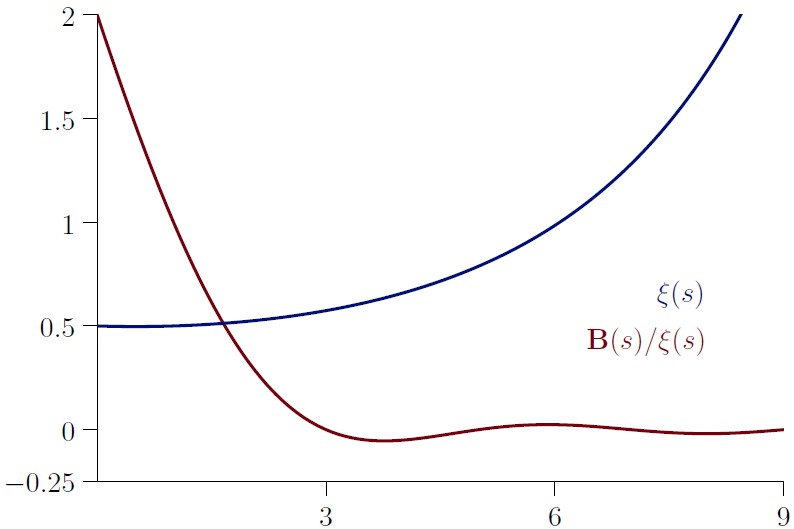The Hadamard decomposition of the Bernoulli function. We denote Hadamard's infinite product over the zeros of $\zeta(s)$ by \begin{equation} \operatorname{H}_{\zeta}(s) \, = \, \frac12\, \prod_{\Im {\rho} > 0 } \left(\left(1 - \frac{s}{\rho} \right)\left(1 - \frac{s}{1- \rho} \right)\right). \label{h99} \end{equation} The product runs over the zeros with $\Im( \rho ) > 0$. The absolute convergence of the product is guaranteed as the terms are taken in pairs $( \rho, 1- \rho )$. Hadamard's infinite product expansion of $\zeta(s)$ is \begin{equation} \zeta(s) \, = \, \frac{\pi^{ s/2 } } {\left(s/2\right)! }\, \frac{\operatorname{H}_{\zeta}(s)}{s-1} \, , \quad s \notin \{1, -2, -4, \dots \}. \label{q55} \end{equation} Applying Jensen's formula (\ref{int1}) leads to the representation \begin{equation} \operatorname{H}_{\zeta}(s) \, = \, \frac{2 (s/2)!}{\pi^{s/2-1}} \int_{- \infty}^\infty\frac{( \tfrac{1}{2} +ix)^{1-s}}{({\mathrm{e}}^{\pi x} + {\mathrm{e}}^ {- \pi x})^2}\,dx. \label{33} \end{equation} Since the zeros of $\zeta(s)$ and $\operatorname{B}(s)$ are identical in the critical strip by (\ref{q52}) this representation carries directly over to the Bernoulli case. Writing $\sigma = (1-s)/2$ we get

\begin{equation} \operatorname{B}(s)\, = \, \frac{\pi^{\sigma}}{\sigma !} \, \operatorname{H}_{\zeta}(s) \, , \quad s \notin \{3,5,7, \dots \}. \label{q56} \end{equation}

This is the Hadamard decomposition of the Bernoulli function. The zeros of $\operatorname{B}(s)$ with $\Im( \rho ) = 0$ are at $3, 5, 7, \ldots$ (making the Bernoulli numbers vanish at these indices), due to the factorial term in the denominator. This representation separates the nontrivial zeros on the critical line from the trivial zeros on the real axes. (See the plot above and for complex $s$ the appendix).

Here we can see another reason why $\operatorname{B}_1 = \frac12$. The oscillating factor has the value $\frac{\pi^0}{0!} = 1$ and the Hadamard factor has the value $\operatorname{H}_{\zeta}(1)= - \zeta(0) \cdot 1$. The Bernoulli value follows from $\zeta(0) = - \frac12$.

If we compare the identities $(\ref{q554})$ and $(\ref{q56})$, we get as a corollary ${ \xi = \operatorname{H}_{\zeta}}.$ This is precisely the proposition that Hadamard proves in his 1893 paper .

### The alternating Bernoulli function

The alternating Bernoulli function is defined as \begin{equation} \operatorname{B}^{*}(s) = \operatorname{B}(s) \left( 1-2^{s} \right). \label{betastar} \end{equation} We can express the alternating Bernoulli function in terms of the zeta function using identity (\ref{q52}) as

\begin{equation} \operatorname{B}^{*}(s) = {s!}\ \zeta(s)\, \tau(s) \left(2^{s} -1 \right). \label{q60} \end{equation}

The alternating Bernoulli numbers are the values of the alternating Bernoulli function at the nonnegative integers, \begin{equation} \operatorname{B}^{*}_n = \operatorname{B}^{*}(n). \label{q61} \end{equation} Like the Bernoulli numbers the alternating Bernoulli numbers are rational numbers. Reduced to lowest terms they have the denominator $2$, $\operatorname{B}^{*}_0 = 0$ and $\operatorname{B}^{*}_1 = - \frac12 .$ In the form $\operatorname{G}_n = 2 \operatorname{B}^{*}_n$ they are the Genocchi numbers introduced above as the values of the Genocchi function at nonnegative integers.

The alternating Riemann zeta function, also known as the Dirichlet eta function, is defined as

\begin{equation} \zeta^{*}(s) = \zeta(s)(1- 2^{1-s}), \quad (s \ne 1). \label{q57} \end{equation} The alternating Bernoulli function can be represented by the alternating zeta function similarly as the Bernoulli function by the zeta function: \begin{equation} \operatorname{B}^{*}(s) = -s \zeta^{*}(1-s), \qquad (s \ne 0). \label{betastardef} \end{equation}

### The alternating Bernoulli polynomials

For a positive real number $x$ define the alternating Hurwitz zeta function as \begin{equation} \zeta^{*}(s, x) \, =\, \sum_{n=0}^{\infty} \frac{(-1)^n}{(n+x)^s}, \quad \text{for } \Re(s) > 0, \label{a61} \end{equation} and for other values of $s$ by analytic continuation. It is connected with the Hurwitz Zeta function by \begin{equation} \zeta^{*}(s, x) \, =\, 2^{-s} \left(\zeta\left(s, \frac{x}{2}\right) - \zeta\left(s, \frac{x+1}{2}\right) \right). \label{b61} \end{equation} The alternating Bernoulli rational polynomials are defined as \begin{equation} \operatorname{B}^{*}_n(x) \, = \, -n \zeta^{*}(1 - n, x) \,. \label{bstar} \end{equation} This definition is in analogy to our introduction of the Bernoulli polynomials (\ref{q22}). The alternating Bernoulli numbers are by (\ref{q123}) half the Genocchi numbers: $\operatorname{B}^{*}_{n} \, = \, 2^{n-1} \left( \operatorname{B}_n\left(\frac{1}{2}\right) - \operatorname{B}_n\left(1 \right) \right) = \operatorname{G}_n/2.$

### The connection with the Euler function

The Euler polynomials are closely related to the Bernoulli polynomials. In handbooks of mathematical functions they are often treated side by side and shown how one can be expressed by the other.

Our perspective is a little different. We recall that the interpolation of rational sequences is our central theme. However, the two cases already differ concerning whether the numbers are values of the respective polynomials: In the Bernoulli case they are, in the Euler case they are not.

The generalized Euler function is defined as a shifted version of the generalized Genocchi function. \begin{align} \operatorname{G}(s, v) \, &= \, 2^s \left(\operatorname{B}\left(s, \frac{v}{2}\right) - \operatorname{B}\left(s, \frac{v+1}{2} \right) \right) \label{GG}, \\ \operatorname{E}(s, v) \, &= \, - \frac{\operatorname{G}(s+1, v)}{s+1} . \label{EG} \end{align} The Euler polynomials are $\operatorname{E}_{n}(x) = \operatorname{E}(n, x)$ for integers $n \ge 0.$ The one-parameter Euler function is the special case $v=1$. \begin{equation} \operatorname{E}(s) \, = \, \operatorname{E}(s, 1) . \label{eulsimpl} \end{equation} The rational numbers $\operatorname{E}(n)$ for integers $n \ge 0$ are \begin{equation} 1,\, \frac12,\, 0,\, - \frac{1}{4},\, 0,\, \frac{1}{2},\, 0,\, - \frac{17}{8},\, 0,\, \frac{31}{2},\, 0,\,- \frac{691}{4},\, 0,\, \frac{5461}{2},\,\ldots . \nonumber \end{equation} However, these numbers do not, differently from the case of the Bernoulli numbers, inherit the name from their defining function. The Euler numbers are the values at the integers of the generalized Euler function (\ref{EG}) at $v= \frac12$, scaled with a power of 2. \begin{equation} \operatorname{E}^{c}(s) \, = \, 2^{s} \operatorname{E}\left(s, \frac{1}{2}\right). \label{EulerCentral} \end{equation} These are the equivalents to the central Bernoulli numbers (\ref{CBN}). Let us gather the two most important special cases of the Euler function. For the Euler polynomials we have

\begin{equation} \operatorname{E}_{n}(x) \, = \, 2 \zeta^{*}(-n, x) \, = \, - 2\, \frac{\operatorname{B}^{*}_{n+1}(x)}{n+1} = \, - \frac{\operatorname{G}_{n+1}(x)}{n+1}. \label{a63} \end{equation}

As a special case we have $2 \operatorname{B}^{*}_n = -n \operatorname{E}_{n-1}(1)$ for $n \ge 1$. For the Euler numbers we have \begin{equation} \operatorname{E}_{n} = 2^n \operatorname{E}_{n}\left( \frac12\right) = -2^{n+1}\frac{\operatorname{B}^{*}_{n+1}\left(\frac12\right)} {n+1} = -2^n \frac{\operatorname{G}_{n+1}(\frac12)}{n+1}. \label{a64} \end{equation}

### The extended Bernoulli numbers

The family tree of the Euler numbers is subdivided into three branches: the Euler secant numbers, the Euler tangent numbers and the André numbers. The André numbers are the secant numbers interwoven with the tangent numbers, that is the term-wise sum of the secant and the tangent sequence.

The traditional way of naming reserves the name Euler numbers for the Euler secant numbers, while the way preferred by combinatorialists  calls the unsigned André numbers Euler numbers. The introduction of the name 'André numbers' in honor of Désiré André who studied their combinatorial interpretation as $2$-alternating permutations in [2, 1879] and [3, 1881] resolves resolves this ambiguity.

 n 0 1 2 3 4 5 6 7 8 $\operatorname{E}_{tan}$ 0 1 0 -2 0 16 0 -272 0 $\operatorname{E}_{sec}$ 1 0 -1 0 5 0 -61 0 1385 $\operatorname{A}$ 1 1 -1 -2 5 16 -61 -272 1385
Let us try to apply the above extension procedure for the Euler numbers to the Bernoulli numbers somehow. First, we name the Bernoulli numbers with even index the Bernoulli tangent numbers and those with odd index (provisionally) the lost Bernoulli numbers.
 n 0 1 2 3 4 5 6 7 8 $\operatorname{B}_{?}$ $1$ ? $\frac{1}{6}$ ? $-\frac{1}{30}$ ? $\frac{1}{42}$ ? $-\frac{1}{30}$
The next table shows what will be the outcome of our choice that we will define in the next section.
 $n$ $0$ $1$ $2$ $3$ $4$ $5$ $6$ $7$ $8$ $\operatorname{B}_{tan}$ $1$ $0$ $\frac{1}{6}$ $0$ $-\frac{1}{30}$ $0$ $\frac{1}{42}$ $0$ $-\frac{1}{30}$ $\operatorname{B}_{sec}$ $0$ $\frac12$ $0$ $-\frac{3}{56}$ $0$ $\frac{25}{992}$ $0$ $-\frac{427}{16256}$ $0$ $\operatorname{B}$ $1$ $\frac12$ $\frac16$ $-\frac{3}{56}$ $-\frac{1}{30}$ $\frac{25}{992}$ $\frac{1}{42}$ $-\frac{427}{16256}$ $-\frac{1}{30}$

### The extended Bernoulli function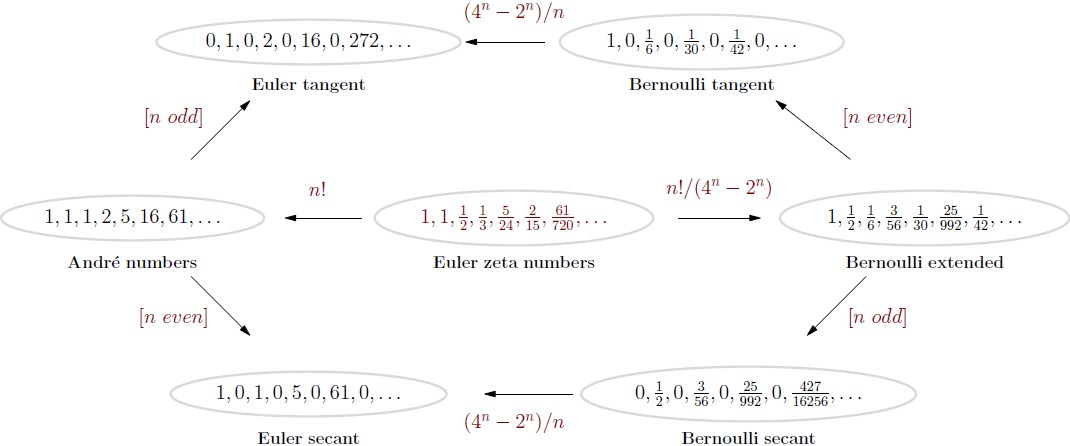The Euler–Bernoulli family of numbers (unsigned version) 🔎 The extended Bernoulli function is defined as

\begin{equation} \mathcal{B}(s) \, = \, -s\, \widetilde{\zeta}(1-s) \label{genbern} \end{equation}

for $s \ne 0$ and the limiting value $\mathcal{B}(0) = 1 + {\pi}/{\log(4)}$, where

\begin{equation} \widetilde{\zeta}(s) \, = \, \zeta \left({s} \right) + \frac{ \zeta \left({s},{\frac{1}{4}} \right) - \zeta \left({s},{\frac{3}{4}} \right) } {{2}^{{s}}-2} . \label{lzeta} \end{equation}

An alternate form to write (\ref{genbern}) is \begin{equation} \mathcal{B}(s) \, = \, \operatorname{B}(s) + s\, \frac{2^{s - 1}}{2^s - 1} \left (\zeta\left(1 - s, \frac14\right) - \zeta\left(1 - s, \frac34\right) \right) . \end{equation} The extended Bernoulli numbers are the values of the extended Bernoulli function at positive integers for $n \ge 2$ and by convention $\operatorname{B}_n= \operatorname{B}(n)$ for $n \in \{0, 1\}$ (see the table above).

The question remains: why did we choose the extended Bernoulli numbers in this manner? The answer is: because of their relation to the Euler zeta numbers, which we will introduce next. The figure above indicates the relations between these six sequences by showing how they derive from a seventh sequence, the Euler zeta numbers.

### The extended Euler function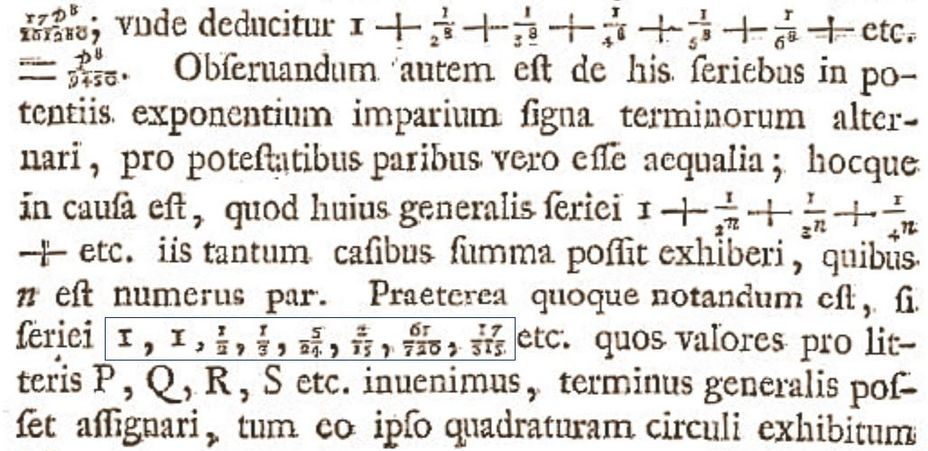L. Euler, De summis serierum reciprocarum, 1735 The Bernoulli numbers and the Euler numbers have a common backbone: the Euler zeta numbers defined as the values at the positive integers of the function \begin{equation} \mathcal{E}(s) \, = \, \frac{4^{s+1} - 2^{s+1}}{ (s+1)!} \mathcal{B}(s+1). \label{gerneul} \end{equation} These numbers, in their unsigned form, were introduced by Leonhard Euler in 1735 in De summis serierum reciprocarum . For $n \ge 0$ they are \begin{equation} 1,\, 1,\, - \frac12,\, - \frac{1}{3},\, \frac{5}{24},\, \frac{2}{15},\, - \frac{61}{720},\, - \frac{17}{315}, \, \frac{277}{8064},\, \frac{62}{2835}, \ldots . \nonumber \end{equation} The André function $\mathcal{A}(s)$ interpolating the André numbers is \begin{align} \mathcal{A}(s) \, &= \, s! \, \mathcal{E}(s) \label{AndreFun} \\ &= (4^{s+1} - 2^{s+1}) \frac{\mathcal{B}(s+1)}{s+1} \\ &= \, (2^{s+1} - 4^{s+1})\, \widetilde{\zeta}(-s). \end{align} When $s$ is a positive integer, these identities are well known, but less known in the generality given here, as identities of complex functions.

### The unsigned extended functions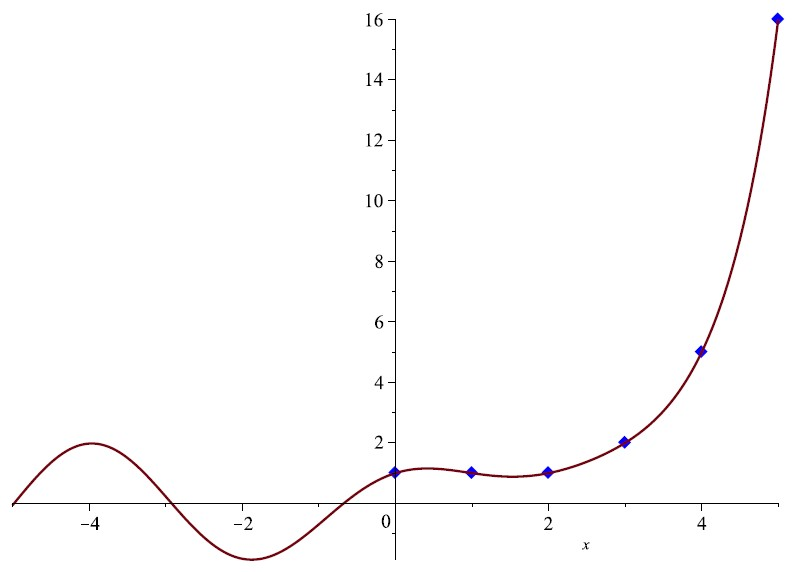The unsigned André function $\mathcal{A}^{\star}$ The unsigned André function has the representation

\begin{equation} \mathcal{A}^{\star} (s) \, = \, i (i^s \operatorname{Li}_{-s}(-i) - (-i)^s \operatorname{Li}_{-s}(i)) \label{UnsignedAndreFun} \end{equation}

where $i$ is the imaginary unit, $\operatorname{Li}_{s}(v)$ is the polylogarithm and the principal branch of the logarithm is used for the powers.

The unsigned André numbers are the values at the nonnegative integers, $\mathcal{A}_n^{\star} = \mathcal{A}^{\star} (n)$. Its counterpart, the unsigned extended Bernoulli function, is defined as

\begin{equation} \mathcal{B}^{\star} (s) \, = \frac{s\, \mathcal{A}^{\star} (s-1)}{4^s - 2^s} \quad (s \ne 0). \label{UnsignedBernoulliFun} \end{equation} For $s = 0$ the function $\mathcal{B}^{\star}$ is supplemented by the limiting value, which, surprisingly, is $-1$. Therefore $\mathcal{B}^{\star}$ interpolates the unsigned extended Bernoulli numbers only for $n \ge 1$.

### The Swiss knife polynomials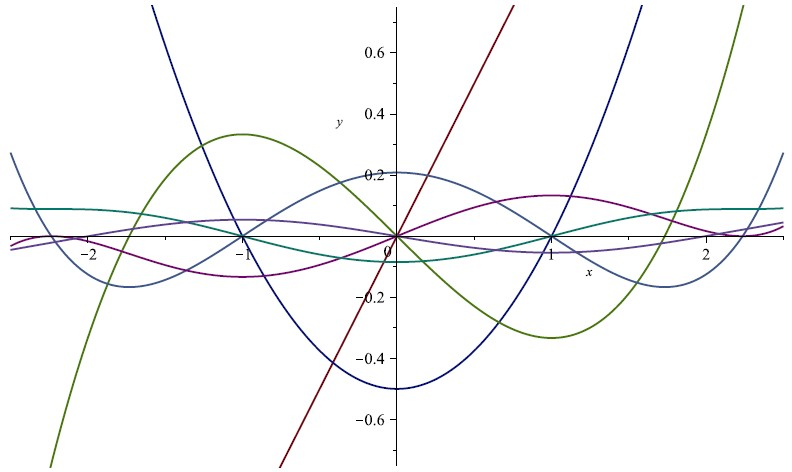The Swiss knife polynomials $\kappa_n(x)/n!$ The reader, exhausted from studying all these higher transcendental functions, may wonder whether the many numbers considered here are also easier to calculate. Fortunately, the answer is yes, and amazingly a single method is sufficient.

\begin{equation} \kappa_n(x) = \sum_{k=0}^{n} \frac{\alpha(k+1)}{2^{ \lfloor k/2 \rfloor}} \sum_{v=0}^{k}(-1)^{v} \binom{k}{v}(x+v+1)^n. \end{equation}

Here $\alpha$ is the repeating sequence period(0, 1, 1, 1, 0, -1, -1, -1). The author dubbed $\kappa_n(x)$ the Swiss knife polynomials because they allow calculating the Euler–Bernoulli family of numbers efficiently. They were introduced in A153641 and discussed in .

The coefficients of the polynomials are integers, in contrast to the coefficients of the Euler and Bernoulli polynomials, which are rational numbers. The polynomials display a beautiful sinusoidal behavior if suitable scaled, which can be explained with the Fourier analysis of the generalized zeta function.

 $1$ $x$ $x^2\,- \, 1$ $x^3\,- \, 3\,x$ $x^4\,- \, 6\,x^2\,+\,5$ $x^5\,- \,10\,x^3\,+\,25\,x$ $x^6\,- \,15\,x^4\,+\,75\,x^2\,- \,61$ $x^7\,- \,21\,x^5\,+\,175\,x^3\,- \,427\,x$ $x^8\,- \,28\,x^6\,+\,350\,x^4\,- \,1708\,x^2\,+\,1385$ $x^9\,- \,36\,x^7\,+\,630\,x^5\,- \,5124\,x^3\,+\,12465\,x$
The Euler, Bernoulli, Genocchi, Euler zeta, tangent as well as the André numbers and the Springer numbers are either values or scaled values of these polynomials, see the table below.
 Euler zeta even $\kappa_n(0)\,/\,n!$ Euler zeta odd $\kappa_n(1)\,/\,n!$ Euler zeta $(-1)^{\lfloor n/2 \rfloor}\, \kappa_n( n \bmod 2)\,/\,n!$ Euler secant $\kappa_n(0)$ Euler tangent $\kappa_n(1)$ Euler extended $(-1)^{\lfloor n/2 \rfloor}\, \kappa_n(n \bmod 2)$ Bernoulli tangent $\kappa_{n-1}(1) \, n \, / \, (4^n - 2^n)$ Bernoulli secant $\kappa_{n-1}(0) \, n \, /\, (4^n - 2^n)$ Bernoulli extended $\kappa_{n-1}((n-1)\, \bmod\, 2)\, n\, /\, (4^n - 2^n)$ Genocchi $\kappa_n( -1) (n+1)\,/\, 2^{n}$ Springer $\kappa_n(1/2)\, 2^n$

### Asymptotics for the Bernoulli function

An asymptotic expansion of the Bernoulli function results directly from $\operatorname{B}(s) = - \zeta(s)\, \tau(s)\, s!$ by using Stirling's formula and the generalized harmonic numbers.

For an even positive integer $n$ the Bernoulli function has an efficient asymptotic approximation ,

\begin{equation} |\operatorname{B}(n)| \, \sim \, 4\,\pi\, \left( \frac {n}{2 \pi \mathrm{e}} \right)^{n+1/2} \exp\left(\frac12 + \frac{n^{-1}}{12} - \frac{n^{-3}}{360} + \frac{n^{-5}}{1260}\right) . \end{equation} Here we use the coefficients in Stirling's expansion for $\log(\Gamma(s))$, see A046969. The number of exact decimal digits guaranteed by this formula is apparently $3 \log(3\, n)$ if $n \ge 50$. This approximation is used in the Boost C++ library  for very large arguments $n$.

For general $s > 0$ the asymptotic expansion is

\begin{align} \begin{split} \operatorname{B}(s) \, &\sim \, -4\, \pi \,\cos \left( \frac{s \pi}{2} \right) \left({\frac { s }{{{2 \pi \mathrm{e}}} }}\right)^{s+1/2} \, \operatorname{R}(s), \\ \operatorname{R}(s) \, &= \, \exp\left(\frac12 + \sum_{n = 1}^{\infty} \frac{\operatorname{B}(n+1)}{n+1} \frac{s^{-n}}{n} \right) . \label{asygen} \end{split} \end{align} If one agrees to the convention to read $\frac{s^{-0}}{0} = 1$, then one can start the sum at $n=0$ and do without the constant term $\frac12$.

Other asymptotic developments can be based on different developments of the Gamma function, for instance on Binet's formula [18, p. 48] generalized by Gergő Nemes [52, 4.2]. More general asymptotic expansions and error bounds follow from those of the Hurwitz zeta function that Nemes established in .

### Epilogue: generating functions

The value of $\operatorname{B}(1)$ deserves special attention. Since it is well known that $\sum_{j=0}^\infty {\gamma_j}/ {j!} = 1/2$ it follows from (\ref{q7}) that $\operatorname{B}(1) = 1/2$. Unfortunately the popular generating function $z/(\mathrm{e}^{z}-1)$ misses this value and disrupts at this point the connection between the Bernoulli numbers and the $\zeta$ function.

For those who do not care about the connection with the zeta function, we add: Even the most elementary relations between the Bernoulli numbers and the Bernoulli polynomials break with this choice. For instance, consider the basic identity (\ref{bernoulligoeshalf}). It applies to all Bernoulli numbers if $\operatorname{B}_n = \operatorname{B}_n(1)$ but not if $\operatorname{B}_n = \operatorname{B}_n(0)$ is set.

Instead use the power series $f(z)$ with constant term 1 such that the coefficient of $x^n$ in $(f(x))^{n+1}$ equals 1 for all $n$. There is only one power series satisfying this condition, as Friedrich Hirzebruch  observes. This series is the Todd function (called after John Arthur Todd)

\begin{equation} \operatorname{T}(z) \,=\, \frac{z}{1- \mathrm{e}^{-z}} \, = \, 1 + \frac{1}{2}\frac{z}{1!} + \frac{1}{6}\frac{z^2}{2!} - \frac{1}{30} \frac{z^4}{4!} + \ldots \end{equation} and generates the Bernoulli numbers matching the values of the Bernoulli function at the nonnegative integers.

A modern exposition based on the Todd series is the monograph . The authors adopt this definition "because it is the original definition of Seki and Bernoulli for one thing, and it is better suited to the special values of the Riemann zeta function for another." Similarly, J. Neukirch in Algebraic Number Theory  writes: The definition $f(z) = z/(1- \mathrm{e}^{-z})$ "is more natural and better suited for the further development of the theory." One might hope that all mathematicians will use this consistent definition one day.

### Acknowledgments

The author thanks Jörg Arndt, Petros Hadjicostas, Václav Kotěšovec, Gergő Nemes and Michael Somos for their reading and providing valuable feedback on an earlier version of this manuscript.

Without using Neil Sloane's OEIS this essay could have been written, but it would only have been half as much fun.

Key words:

Bernoulli function, Bernoulli constants, Bernoulli numbers, Bernoulli cumulants, Bernoulli functional equation, Bernoulli central function, extended Bernoulli function, Bernoulli central polynomials, alternating Bernoulli function, Stieltjes constants, Riemann zeta function, Hurwitz zeta function, Worpitzky numbers, Worpitzky transform, Hasse representation, Hadamar product, Genocchi numbers, André numbers, Swiss knife polynomials.

2020 MSC. Primary 11B68, Secondary 11M35.

Code repository and supplements, GitHub .

### References

• T. M. Apostol, Zeta and related functions, chapter 25 of the Digital Library of Mathematical Functions (DLMF), release 1.0.18 of 2018-03-27, →DLMF.
• D. André, Développement de $\sec\, x$ and $\mathrm{tg}\, x$, C. R. Math. Acad. Sci. Paris (88), 965–979, (1879).
• D. André, Mémoire sur les permutations alternées, J. Math. pur. appl. (7), 167–184, (1881).
• T. M. Apostol, Introduction to analytic number theory, Springer, 1976.
• T. Arakawa, T. Ibukiyama, M. Kaneko, Bernoulli numbers and zeta functions, Springer, 2014.
• R. Ayoub, Euler and the zeta function, Amer. Math. Monthly, 81 No.10, 1067–1086, (1974).
• Boost C++ libraries, Version 1.73.0, April 2020, →Boost.
• K. Chakraborty, S. Kanemitsu and H. Tsukada, Vistas of Special Functions II, World Scientific Publishing, 2009.
• L. Comtet, Advanced Combinatorics, Reidel Publishing, 1974.
• D. F. Connon, Some series and integrals involving the Riemann zeta function, binomial coefficients and the harmonic numbers. Volume II(b), arXiv:0710.4024v2 [math.HO], (2007).
• D. Cvijović, A note on convexity properties of functions related to the Hurwitz zeta and alternating Hurwitz zeta function, Journal of Mathematical Analysis and Applications, 487(1), (2020).
• I. V. Blagouchine, A theorem for the closed-form evaluation of the first generalized Stieltjes constant at rational arguments and some related summations, Journal of Number Theory, vol. 148, pp. 537–592 and vol. 151, pp. 276–277, (2015).
• I. V. Blagouchine, Expansions of generalized Euler's constants into the series of polynomials in $\pi^{-2}$ and into the formal enveloping series with rational coefficients only, Journal of Number Theory, vol. 158, pp. 365–396, (2016).
• I. V. Blagouchine, Three Notes on Ser's and Hasse's Representations for the Zeta-Functions, Integers, Electronic Journal of Combinatorial Number Theory, vol. 18A, article #A3, pp. 1–45, (2018).
• H. M. Edwards, Riemann's Zeta Function, Academic Press, 1974.
• N. Elezovic, Asymptotic Expansions of Central Binomial Coefficients and Catalan Numbers, J. Int. Seq. 17 (2014).
• N. D. Elkies, On the sums $\sum_{k=- \infty}^{\infty} (4k + 1)^{-n}$, Amer. Math. Monthly (110), 561–573, (2003).
• A. Erdélyi, W. Magnus, F. Oberhettinger, and F. Tricomi, Higer Transcendental Functions, Vol. I, NewYork, 1953.
• L. Euler, On the sums of series of reciprocals, p.5. →E41-English
• L. Euler, De summis serierum reciprocarum, Commentarii academiae scientiarum Petropolitanae 7 (1740), 12–134. →E41
• L. Euler, Institutiones calculi differentialis, St. Petersburg Academy of Sciences, 1755. Reprinted in the Opera Omnia, Series 1, vol 10. →E212
• L. Euler, De summis serierum numeros Bernoullianos involventium, Novi Commentarii academiae scientiarum Petropolitanae, Volume 14, pp. 129-167, 1768/1770. Reprinted in Opera Omnia, Series 1 vol 15, pp. 91-130, →E393.
• J. Franel, Note no. 245, L'Intermédiaire des mathématiciens, tome II, pp. 153–154, (1895).
• R. L. Graham, D. E. Knuth, and O. Patashnik, Concrete Mathematics: A Foundation for Computer Science, Addison-Wesley, 1989.
• J. Hadamard, Étude sur les propriétés des fonctions entières et en particulier d'une fonction considérée par Riemann, Journal de Mathématiques Pures et Appliquées (58), 171–216, (1893).
• H. Hasse, Ein Summierungsverfahren für die Riemannsche $\zeta$-Reihe, Math. Z. (32), pp. 458–464, (1930).
• J. Havil, Gamma, Exploring Euler's Constant, Princeton University Press, (2017).
• F. Hirzebruch, The signature theorem: Reminiscences and recreation, In: Prospects in mathematics. Annals of mathematics studies, (70), pp. 3–31, (1971).
• P. C. Hu, C. C. Yang, Value Distribution Theory Related to Number Theory, Birkhäuser, (2006).
• A. Hurwitz, Einige Eigenschaften der Dirichlet'schen Functionen $F(s)=\sum (D/n)\cdot 1/n^s$, die bei der Bestimmung der Classenanzahlen binärer quadratischer Formen auftreten, Zeitschr. Math. Phys. 27, 86–101, (1882).
• J. L. W. V. Jensen, Remarques relatives aux réponses de MM. Franel et Kluyver. L'Intermédiaire des mathématiciens, tome II, Gauthier-Villars et Fils, pp. 346–347, (1895).
• F. Johansson, Rigorous high-precision computation of the Hurwitz zeta function and its derivatives, Numer Algor 69, 253–270 (2015).
• F. Johansson and I. V. Blagouchine, Computing Stieltjes constants using complex integration, Mathematics of Computation, 88:318 (2019), 1829–1850
• B. C. Kellner, J. Sondow, The denominators of power sums of arithmetic progressions, arXiv:1705.05331, 2017; Integers 18, Article A95, (2018).
• W. Lang, On Sums of Powers of Arithmetic Progressions, and Generalized Stirling, Eulerian and Bernoulli numbers, arXiv:1707.04451, (2017).
• J. C. Lagarias, Euler's constant: Euler's work and modern developments, arXiv:1303.1856, (2013).
• D. H. Lehmer, A new approach to Bernoulli polynomials, The American Mathematical Monthly 95.10, 905–911, (1988).
• E. Lindelöf, Le calcul des résidus et ses applications à l'théorie des fonctions, Gauthier-Villars, Paris, 1905.
• LMFDB, The L-functions and Modular Forms Database, →LMFDB.
• Peter H. N. Luschny, An Introduction to the Bernoulli function, →arXiv:2009.06743 [math.HO], (2020).
• P. Luschny, The Bernoulli Manifesto, online since 2013, →Manifesto.
• P. Luschny, Generalized Bernoulli Numbers, (2013), →GeneralizedBN.
• P. Luschny, A sequence transformation and the Bernoulli numbers, (2010), →SeqTrans.
• P. Luschny, Computation and asymptotics of Bernoulli numbers, (2012), →CompBern.
• P. Luschny, Eulerian polynomials, →EulPoly.
• P. Luschny, Supplements for 'An Introduction to the Bernoulli Function', (2020), →Supp.
• P. Luschny, The Swiss-Knife polynomials and Euler numbers, (2010), →SKPoly.
• P. Luschny, The lost Bernoulli numbers, (2011), →LostBN.
• D. Merlini, R. Sprugnoli, M. C. Verri, The Akiyama-Tanigawa transformation, Integers 5/A05, (2005).
• B. Mazur, Bernoulli numbers and the unity of mathematics, manuscript, →Unity.
• J. Musser, Higher derivatives of the Hurwitz zeta function, Masters Theses and Specialist Projects, (2011), →DerivHurwitz.
• G. Nemes, Generalization of Binet's Gamma function formulas, Integral Transforms and Special Functions, 24:8, 597–606, (2013).
• G. Nemes, Error bounds for the asymptotic expansion of the Hurwitz zeta function, Proceedings of the Royal Society A: Mathematical, Physical and Engineering Sciences 473, (2017).
• J. Neukirch, Algebraic Number Theory, Springer, 1999.
• J. L. Raabe, Zurückführung einiger Summen und bestimmten Integrale auf die Jacob-Bernoullische Function, J. Reine Angew. Math. (42), 348–367, (1851).
• B. Riemann, On the number of primes less than a given magnitude, (1859), →NumOfPrimes.
• C. Sandifer, Some Facets of Euler's Work on Series. Studies in the History and Philosophy of Mathematics (5), (2007). Also in: Leonhard Euler: Life, Work and Legacy, R. E. Bradley and E. Sandifer (Ed.), Elsevier, 2007.
• N. J. A. Sloane et al., The On-Line Encyclopedia of Integer Sequences, →OEIS.
• R. P. Stanley, A survey of alternating permutations, Contemp. Math. (531), pp. 165–196, (2010).
• J. Stopple (Q), H. Cohen (A), F. Johansson (A), On a certain integral representation for Hurwitz zeta functions, (2018), →IntRep.
• H. M. Srivastava, J. Choi, Series Associated with the Zeta and Related Functions, Springer, 2001
• T. Tao, The Euler-Maclaurin formula, Bernoulli numbers, the zeta function, and real-variable analytic continuation, (2010), →EulerMac.
• T. Tao, Comment on $\tau$. →TauNum.
• S. Vandervelde, The Worpitzky Numbers Revisited, The American Mathematical Monthly, 125:3, pp. 198–206, (2018).
• A. Voros, Zeta Functions over Zeros of Zeta Functions, Lecture Notes of the Unione Matematica Italiana Vol 8, 2010.
• A. Weil, Number theory: an approach through history. From Hammurapi to Legendre, Birkhäuser, 1983.
• H. S. Wilf, generatingfunctionology, Academic Press, 1990.
• S. C. Woon, Analytic continuation of Bernoulli numbers, a new formula for the Riemann zeta function, and the phenomenon of scattering of zeros. Preprint DAMTP-R-97/19, arXiv:physics/9705021.
• J. Worpitzky, Studien über die Bernoullischen und Eulerschen Zahlen, Journal für die reine und angewandte Mathematik (94), pp. 203–232, (1883).

### Bernoulli constants

 $n$ $\beta_n$ $\beta_n /n!$ 0 +1.00000000000000000000000e+00 +1.00000000000000000000000e+00 1 -5.77215664901532860606512e-01 -5.77215664901532860606512e-01 2 +1.45631690967353449721173e-01 +7.28158454836767248605864e-02 3 +2.90710895786169554535912e-02 +4.84518159643615924226519e-03 4 -8.21533768121338346464019e-03 -3.42305736717224311026674e-04 5 -1.16268503273365002873409e-02 -9.68904193944708357278404e-05 6 -4.75994290380637621052001e-03 -6.61103181084218918127779e-06 7 +1.67138541801139726910695e-03 +3.31624090875277235933919e-07 8 +4.21831653646200836859278e-03 +1.04620945844791874221051e-07 9 +3.16911018422735558641847e-03 +8.73321810027379736116201e-09 10 +3.43947744180880481779146e-04 +9.47827778276235895555407e-11 11 -2.25866096399971274152095e-03 -5.65842192760870796637242e-11 12 -3.24221327452684232007482e-03 -6.76868986351369665586675e-12 13 -2.17454785736682251359552e-03 -3.49211593667203185445522e-13 14 +3.84493292452642224040106e-04 +4.41042474175775338023724e-15 15 +3.13813893088949918755710e-03 +2.39978622177099917550506e-15 16 +4.53549848512386314628695e-03 +2.16773122007268285496389e-16 17 +3.39484659125248617003234e-03 +9.54446607636696517342499e-18 18 -4.72986667978530060590399e-04 -7.38767666053863649781558e-20 19 -5.83999975483580370526234e-03 -4.80085078248806522761766e-20 20 -1.00721090609471125811119e-02 -4.13995673771330564126948e-21 21 -9.79321479174274843741249e-03 -1.91682015939912339496482e-22 22 -2.29763093463200254783750e-03 -2.04415431222621660772759e-24 23 +1.24567903906919471380695e-02 +4.81849850110735344392922e-25 24 +2.98550901697978987031938e-02 +4.81185705151256647946111e-26 25 +3.97127819725890390476549e-02 +2.56026331031881493660913e-27 26 +2.79393907712007094428316e-02 +6.92784089530466712388013e-29 27 -1.77336950032031696506289e-02 -1.62860755048558674407104e-30 28 -9.73794335813190698522061e-02 -3.19393756115325557604211e-31 29 -1.85601987419318254285110e-01 -2.09915158936342552768549e-32 30 -2.21134553114167174032372e-01 -8.33674529544144047562508e-34 31 -1.10289594522767989385320e-01 -1.34125937721921866750473e-35 32 +2.40426431930087325860325e-01 +9.13714389129817199794565e-37 33 +8.48223060577873259185100e-01 +9.76842144689316562821221e-38 34 +1.53362895967472747676942e+00 +5.19464288745573322360277e-39 35 +1.78944248075279625487765e+00 +1.73174959516100441594633e-40 36 +7.33429569739007257407068e-01 +1.97162023326628724184554e-42 37 -2.68183987622201934815062e+00 -1.94848008275558832944550e-43 38 -8.96900252642345710339731e+00 -1.71484028164349789185891e-44 39 -1.67295744090075569567376e+01 -8.20162325795024844398325e-46 40 -2.07168737077169487591557e+01 -2.53909617003982347034561e-47 41 -1.01975839351792340684642e+01 -3.04837480681247325379474e-49 42 +3.02221435698546147289327e+01 +2.15103288078139524274228e-50 43 +1.13468181875436585038896e+02 +1.87813767783170614584043e-51 44 +2.31656933743621048467685e+02 +8.71456987091534575015368e-53 45 +3.23493565027658727705394e+02 +2.70429325494165277375631e-54 46 +2.33327851136153134645751e+02 +4.24030297482975157602467e-56 47 -3.10666033627557393250479e+02 -1.20123014394335413203436e-57 48 -1.63390919914361991588774e+03 -1.31619164554817482023699e-58 49 -3.85544150839888666284589e+03 -6.33824832920169119558284e-60 50 -6.29221938159892345466820e+03 -2.06884990450560309799972e-61
 $n$ $\mathcal{B}_n^{\star}$ $\mathcal{E}_n^{\star}$ $0$ $1$ $1$ $1$ ${\frac{1}{2}}$ $1$ $2$ ${\frac{1}{6}}$ ${\frac{1}{2}}$ $3$ ${\frac{3}{56}}$ ${\frac{1}{3}}$ $4$ ${\frac{1}{30}}$ ${\frac{5}{24}}$ $5$ ${\frac{25}{992}}$ ${\frac{2}{15}}$ $6$ ${\frac{1}{42}}$ ${\frac{61}{720}}$ $7$ ${\frac{427}{16256}}$ ${\frac{17}{315}}$ $8$ ${\frac{1}{30}}$ ${\frac{277}{8064}}$ $9$ ${\frac{12465}{261632}}$ ${\frac{62}{2835}}$ $10$ ${\frac{5}{66}}$ ${\frac{50521}{3628800}}$ $11$ ${\frac{555731}{4192256}}$ ${\frac{1382}{155925}}$ $12$ ${\frac{691}{2730}}$ ${\frac{540553}{95800320}}$ $13$ ${\frac{35135945}{67100672}}$ ${\frac{21844}{6081075}}$ $14$ ${\frac{7}{6}}$ ${\frac{199360981}{87178291200}}$ $15$ ${\frac{2990414715}{1073709056}}$ ${\frac{929569}{638512875}}$ $16$ ${\frac{3617}{510}}$ ${\frac{3878302429}{4184557977600}}$ $17$ ${\frac{329655706465}{17179738112}}$ ${\frac{6404582}{10854718875}}$ $18$ ${\frac{43867}{798}}$ ${\frac{2404879675441}{6402373705728000}}$ $19$ ${\frac{45692713833379}{274877382656}}$ ${\frac{443861162}{1856156927625}}$ $20$ ${\frac{174611}{330}}$ ${\frac{14814847529501}{97316080327065600}}$ $21$ ${\frac{1111113564712575}{628292059136}}$ ${\frac{18888466084}{194896477400625}}$ $22$ ${\frac{854513}{138}}$ ${\frac{69348874393137901}{1124000727777607680000}}$ $23$ ${\frac{1595024111042171723}{70368735789056}}$ ${\frac{113927491862}{2900518163668125}}$ $24$ ${\frac{236364091}{2730}}$ ${\frac{238685140977801337}{9545360026665222144000}}$ $25$ ${\frac{387863354088927172625}{1125899873288192}}$ ${\frac{58870668456604}{3698160658676859375}}$ $26$ ${\frac{8553103}{6}}$ ${\frac{4087072509293123892361}{403291461126605635584000000}}$ $27$ ${\frac{110350957750914345093747}{18014398375264256}}$ ${\frac{8374643517010684}{1298054391195577640625}}$ $28$ ${\frac{23749461029}{870}}$ ${\frac{13181680435827682794403}{3209350995912777478963200000}}$ $29$ ${\frac{36315529600705266098580265}{288230375614840832}}$ ${\frac{689005380505609448}{263505041412702261046875}}$ $30$ ${\frac{8615841276005}{14322}}$ ${\frac{441543893249023104553682821}{265252859812191058636308480000000}}$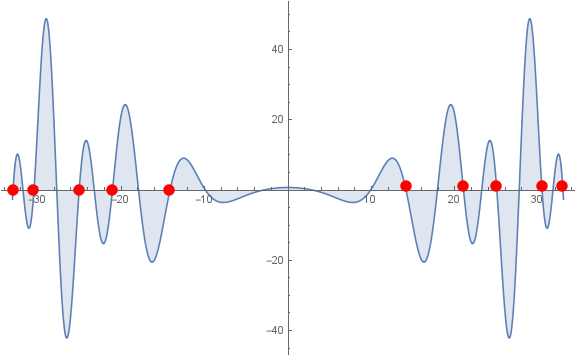Bernoulli function and Riemann zeros on the critical line.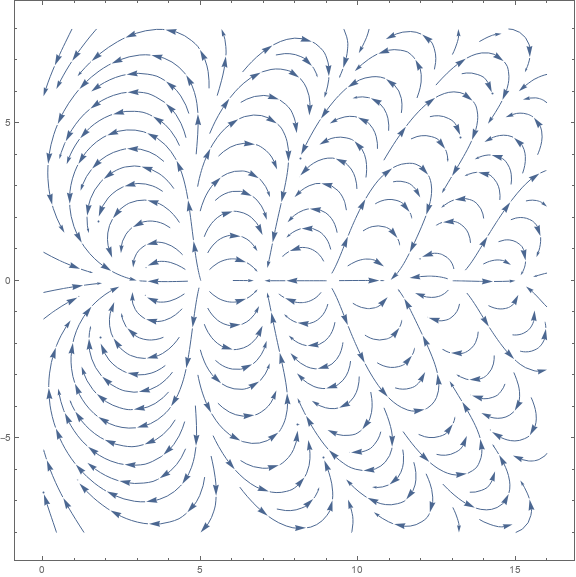Phase portrait of $\operatorname{B}(x)$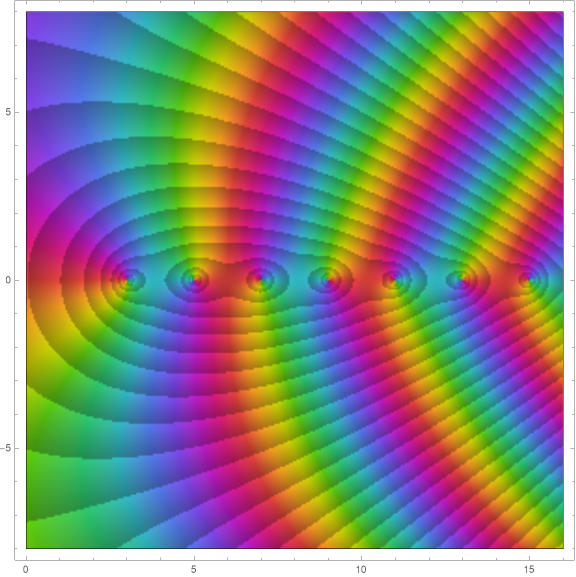Complex view of $\operatorname{B}(x)$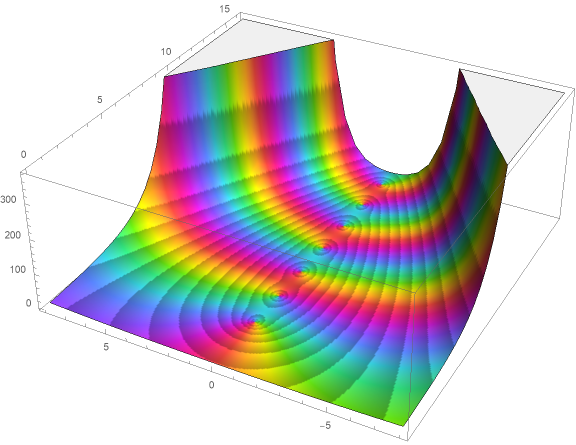3-dim view of $\operatorname{B}(x)$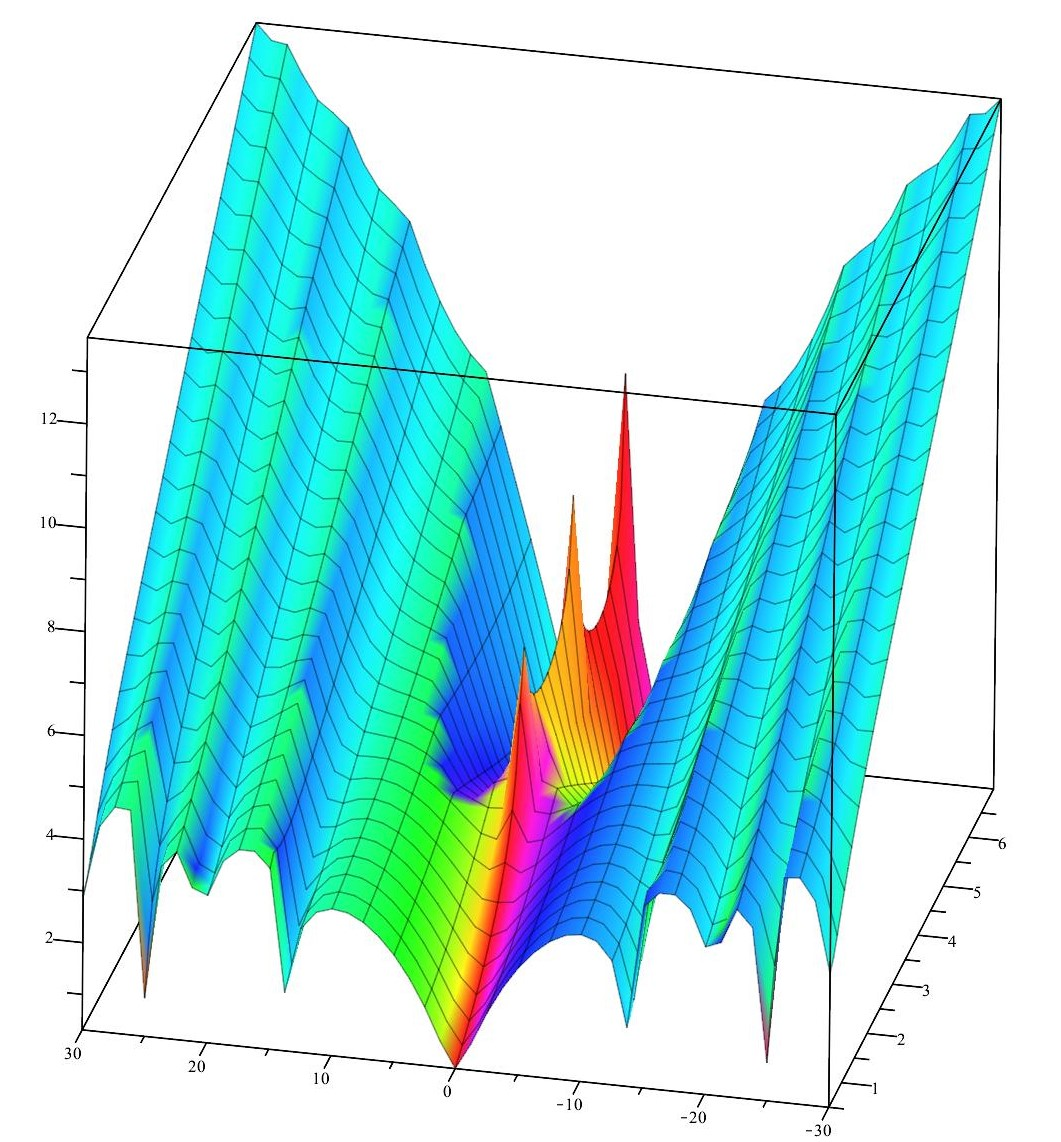Logarithm of $\operatorname{B}(x)$ on the right half plane. The red peaks on the x-axis correspond to the real zero of the Bernoulli function (the vanishing of the odd Bernoulli numbers). The front side of the plot shows the logarithm of the Bernoulli function on the critical line.The Hadamard decomposition of $\log \operatorname{B}:$ the Riemann $\xi$-factor.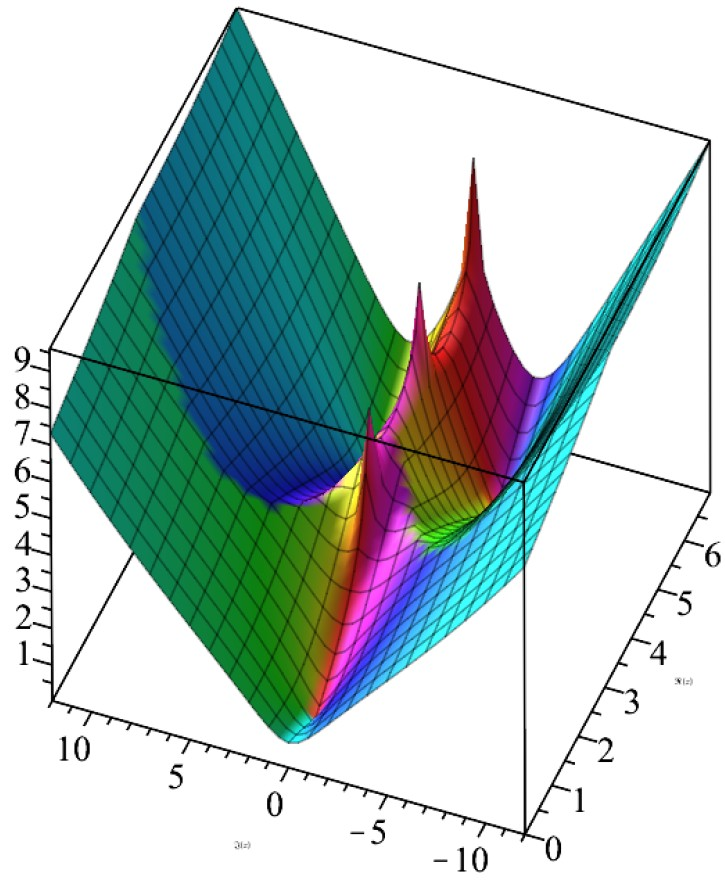The Hadamard decomposition of $\log \operatorname{B}:$ the singularity factor.